Скачать презентацию CS 155 Spring 2010 Cryptography Overview John Mitchell

2335a177040dd36de95ec4fe8196d131.ppt

• Количество слайдов: 87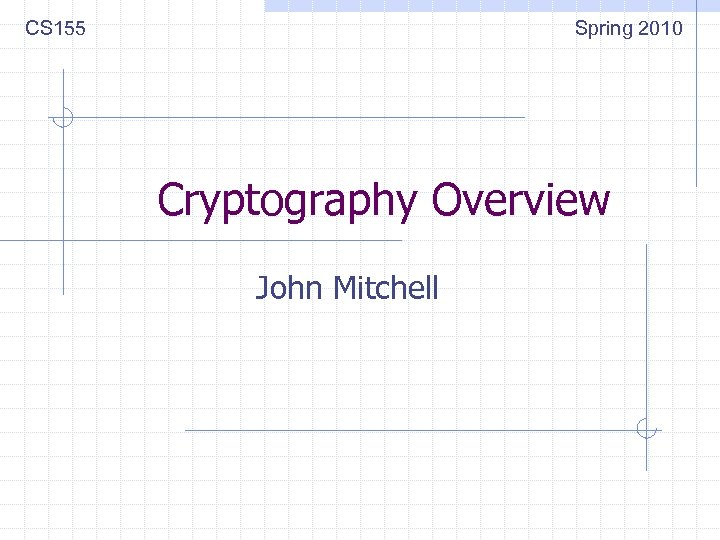CS 155 Spring 2010 Cryptography Overview John Mitchell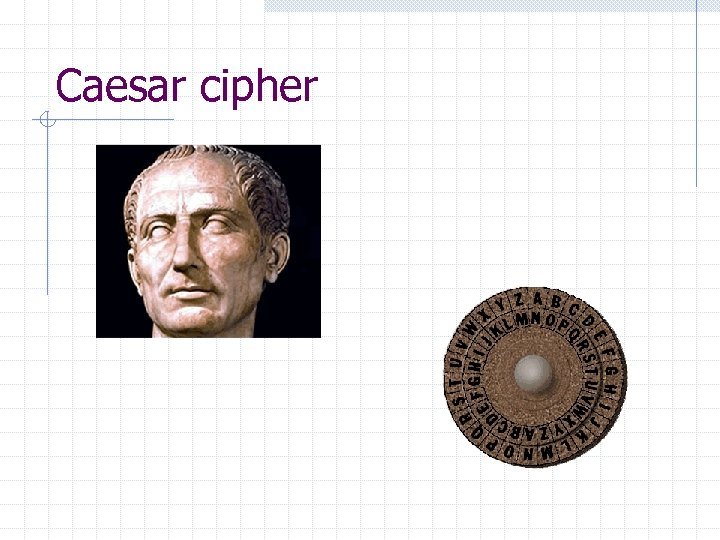Caesar cipher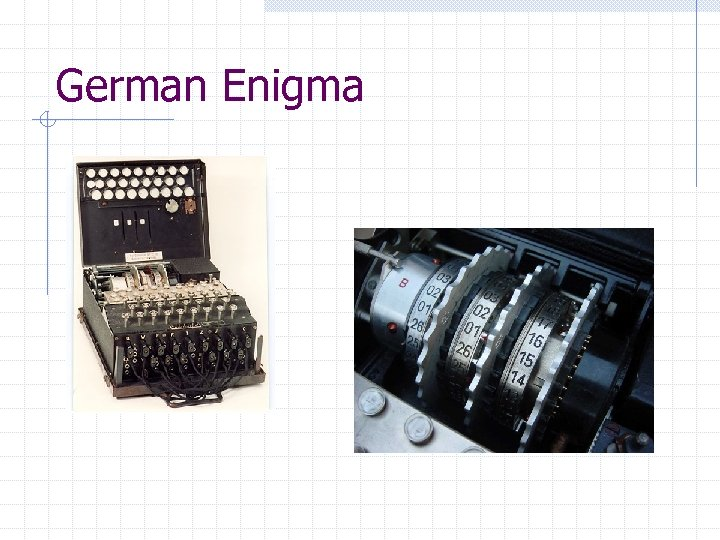German Enigma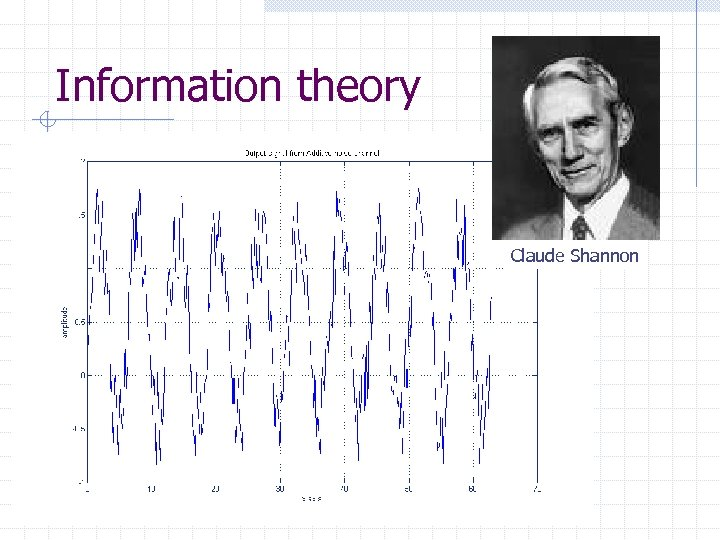Information theory Claude Shannon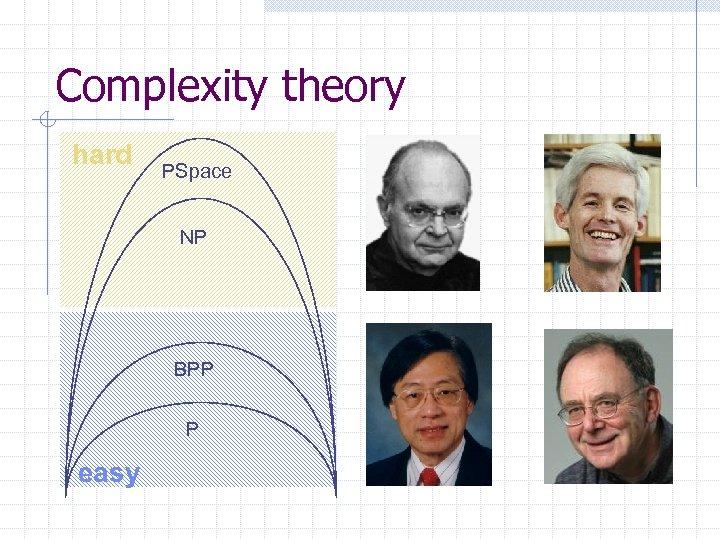Complexity theory hard PSpace NP BPP P easy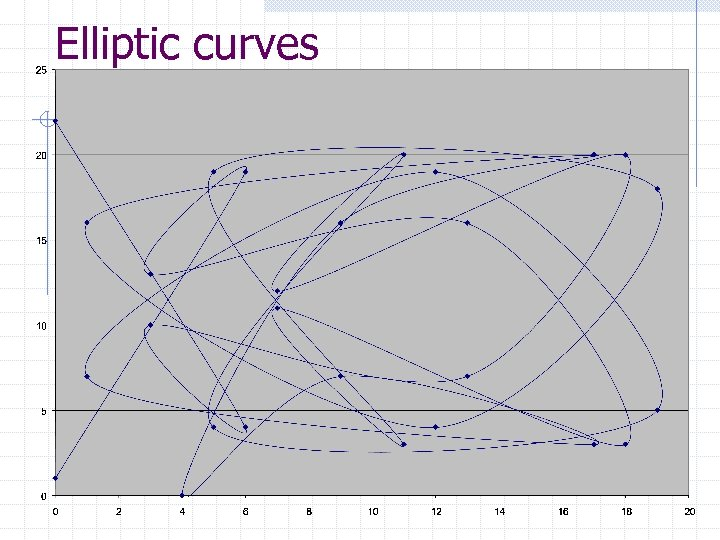Elliptic curves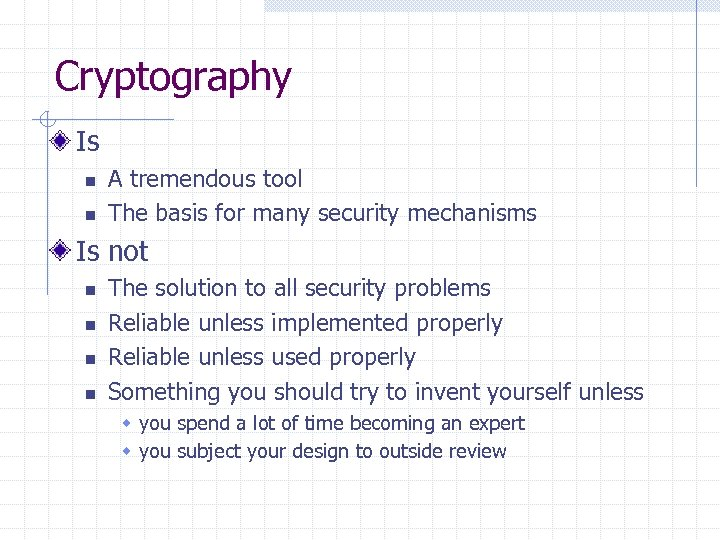Cryptography Is n n A tremendous tool The basis for many security mechanisms Is not n n The solution to all security problems Reliable unless implemented properly Reliable unless used properly Something you should try to invent yourself unless w you spend a lot of time becoming an expert w you subject your design to outside review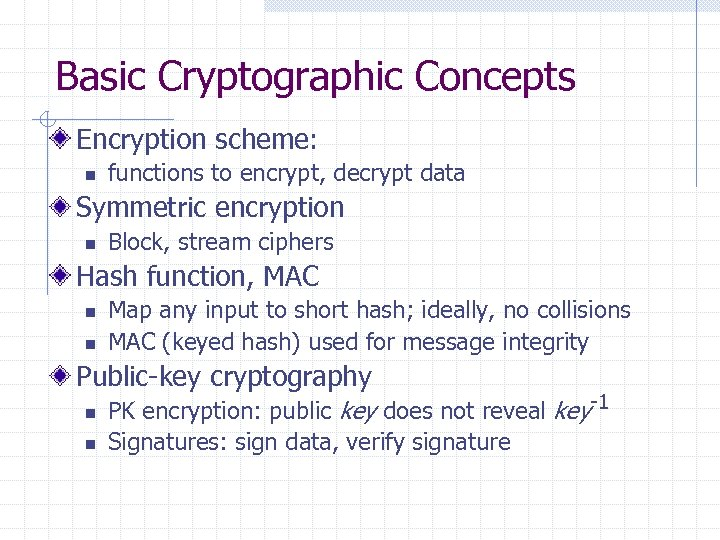Basic Cryptographic Concepts Encryption scheme: n functions to encrypt, decrypt data Symmetric encryption n Block, stream ciphers Hash function, MAC n n Map any input to short hash; ideally, no collisions MAC (keyed hash) used for message integrity Public-key cryptography n n PK encryption: public key does not reveal key-1 Signatures: sign data, verify signature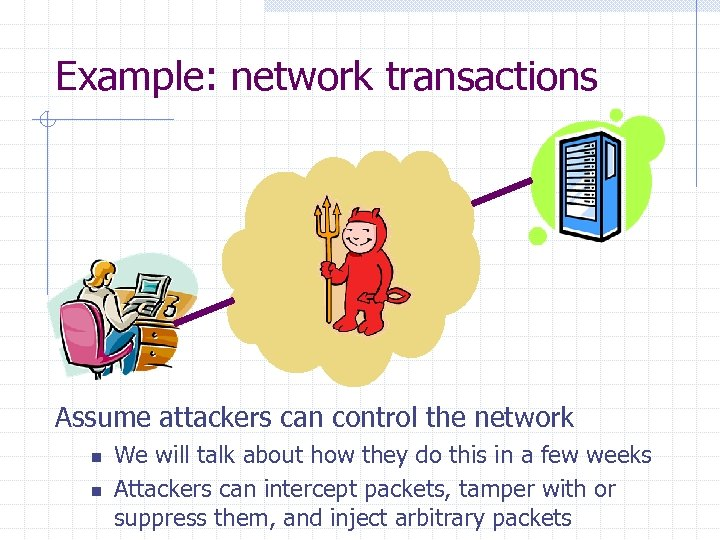Example: network transactions Assume attackers can control the network n n We will talk about how they do this in a few weeks Attackers can intercept packets, tamper with or suppress them, and inject arbitrary packets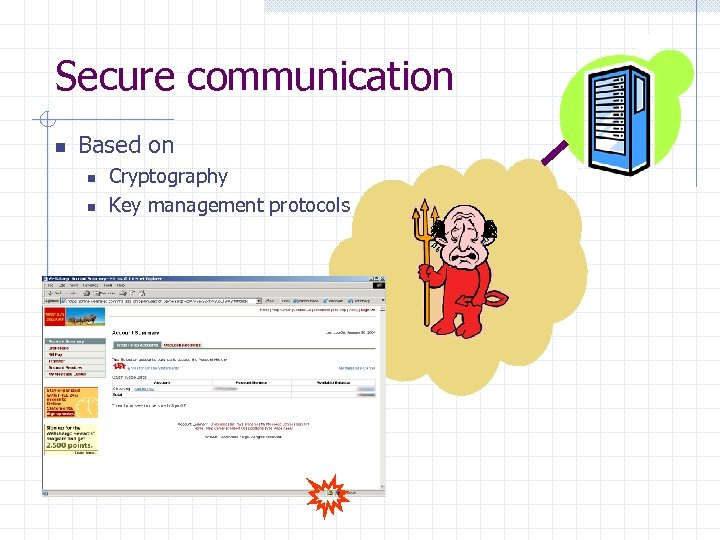Secure communication n Based on n n Cryptography Key management protocols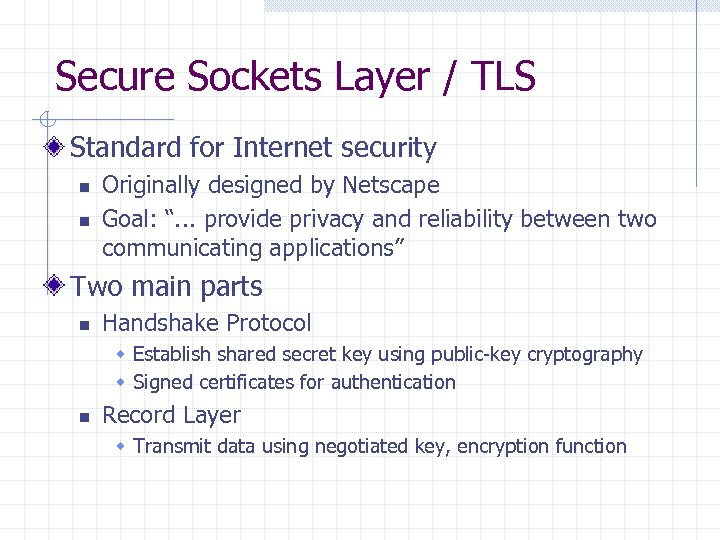Secure Sockets Layer / TLS Standard for Internet security n n Originally designed by Netscape Goal: “. . . provide privacy and reliability between two communicating applications” Two main parts n Handshake Protocol w Establish shared secret key using public-key cryptography w Signed certificates for authentication n Record Layer w Transmit data using negotiated key, encryption function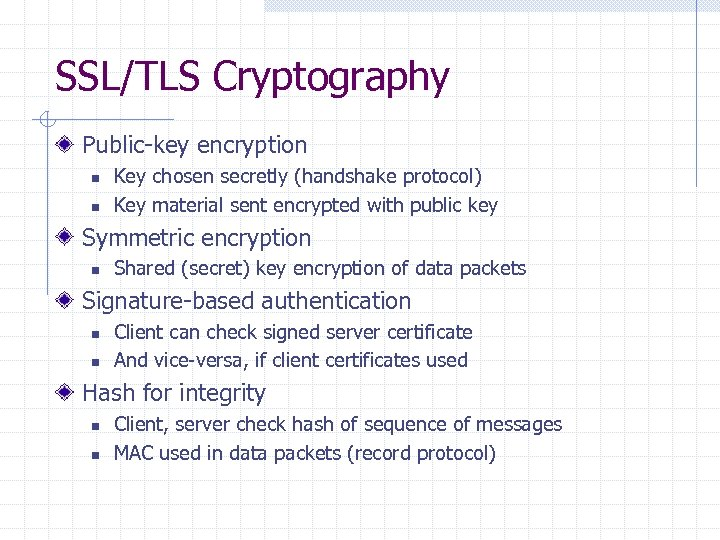SSL/TLS Cryptography Public-key encryption n n Key chosen secretly (handshake protocol) Key material sent encrypted with public key Symmetric encryption n Shared (secret) key encryption of data packets Signature-based authentication n n Client can check signed server certificate And vice-versa, if client certificates used Hash for integrity n n Client, server check hash of sequence of messages MAC used in data packets (record protocol)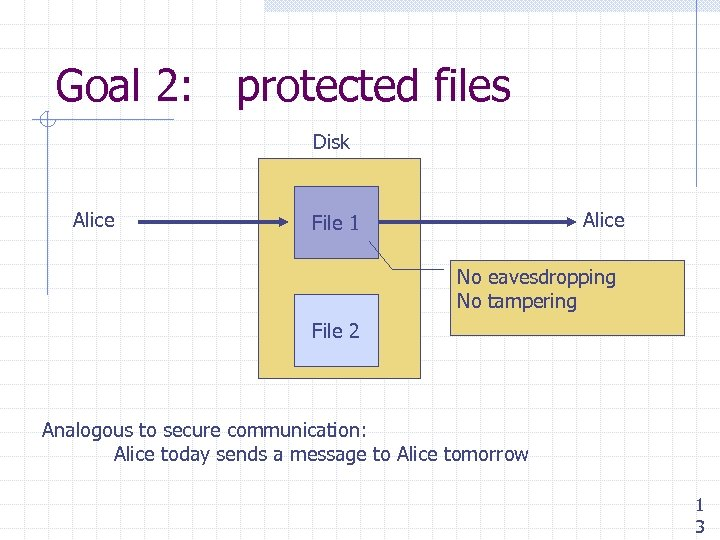Goal 2: protected files Disk Alice File 1 No eavesdropping No tampering File 2 Analogous to secure communication: Alice today sends a message to Alice tomorrow 1 3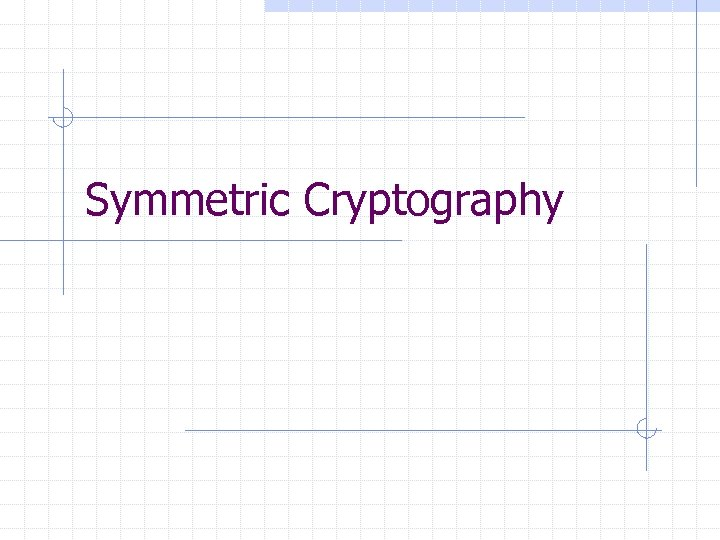Symmetric Cryptography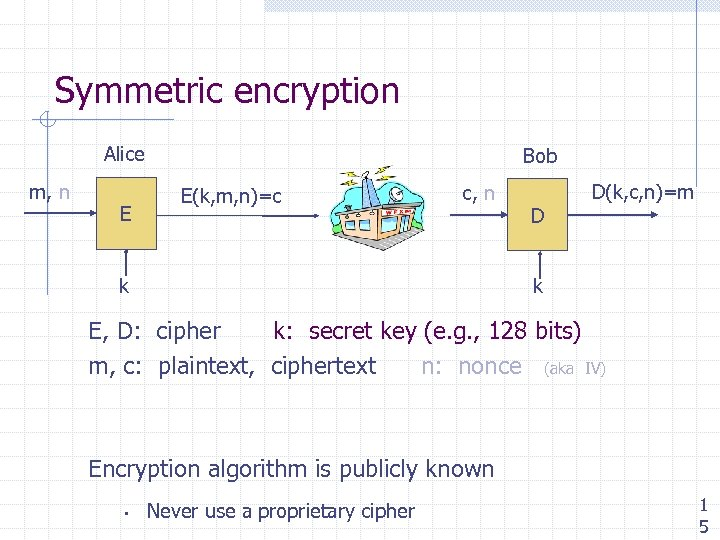Symmetric encryption Alice m, n E Bob E(k, m, n)=c c, n D D(k, c, n)=m k k E, D: cipher k: secret key (e. g. , 128 bits) m, c: plaintext, ciphertext n: nonce (aka IV) Encryption algorithm is publicly known • Never use a proprietary cipher 1 5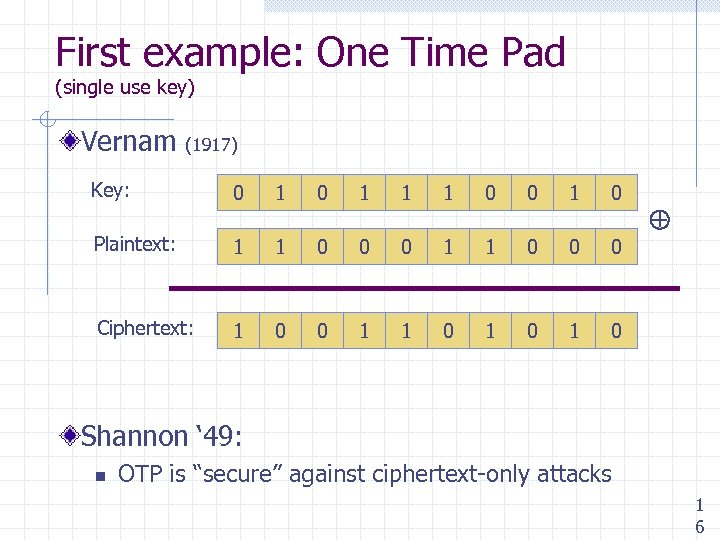First example: One Time Pad (single use key) Vernam (1917) Key: 0 1 1 1 0 0 1 0 Plaintext: 1 1 0 0 0 Ciphertext: 1 0 0 1 1 0 1 0 Shannon ‘ 49: n OTP is “secure” against ciphertext-only attacks 1 6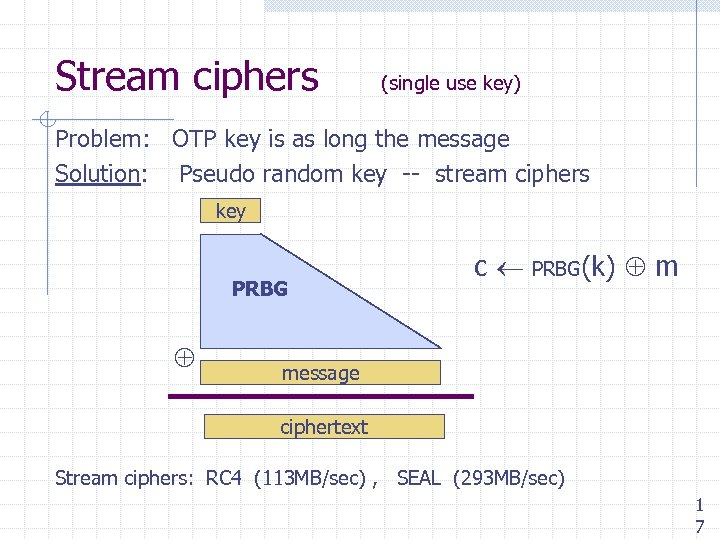Stream ciphers (single use key) Problem: OTP key is as long the message Solution: Pseudo random key -- stream ciphers key PRBG c PRBG(k) m message ciphertext Stream ciphers: RC 4 (113 MB/sec) , SEAL (293 MB/sec) 1 7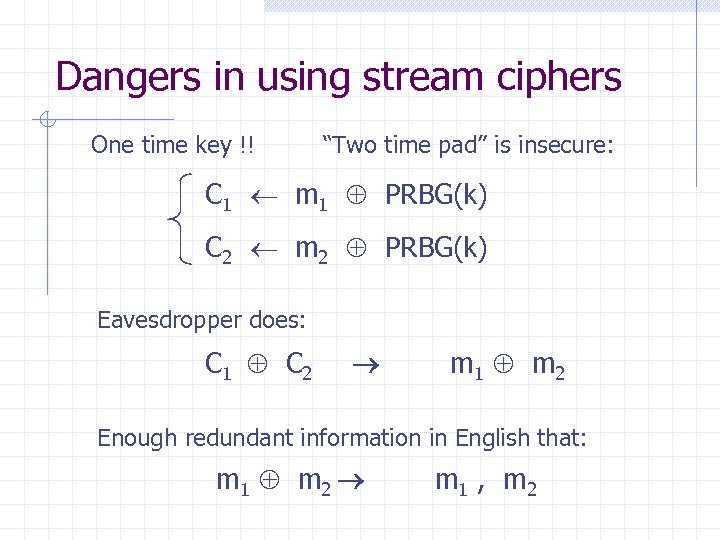Dangers in using stream ciphers One time key !! “Two time pad” is insecure: C 1 m 1 PRBG(k) C 2 m 2 PRBG(k) Eavesdropper does: C 1 C 2 m 1 m 2 Enough redundant information in English that: m 1 m 2 m 1 , m 2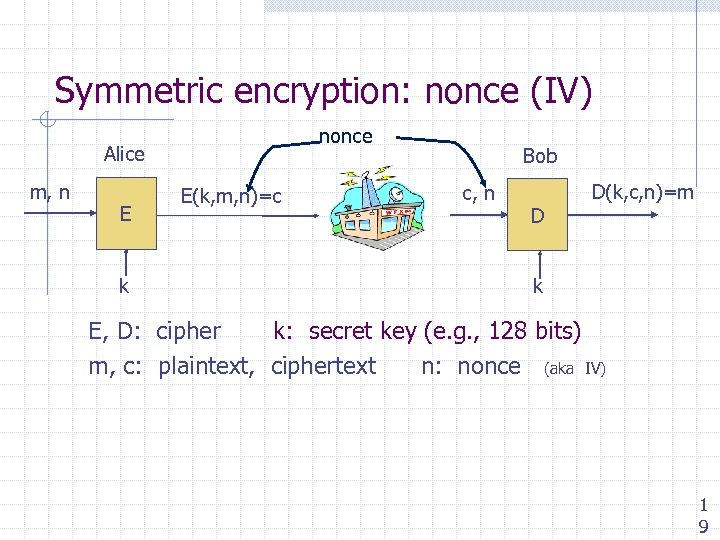Symmetric encryption: nonce (IV) nonce Alice m, n E k E(k, m, n)=c Bob c, n D D(k, c, n)=m k E, D: cipher k: secret key (e. g. , 128 bits) m, c: plaintext, ciphertext n: nonce (aka IV) 1 9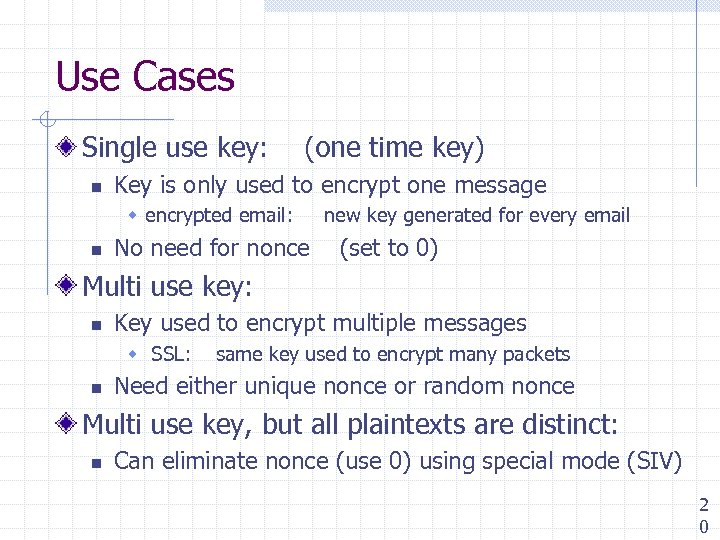Use Cases Single use key: n (one time key) Key is only used to encrypt one message w encrypted email: n No need for nonce new key generated for every email (set to 0) Multi use key: n Key used to encrypt multiple messages w SSL: n same key used to encrypt many packets Need either unique nonce or random nonce Multi use key, but all plaintexts are distinct: n Can eliminate nonce (use 0) using special mode (SIV) 2 0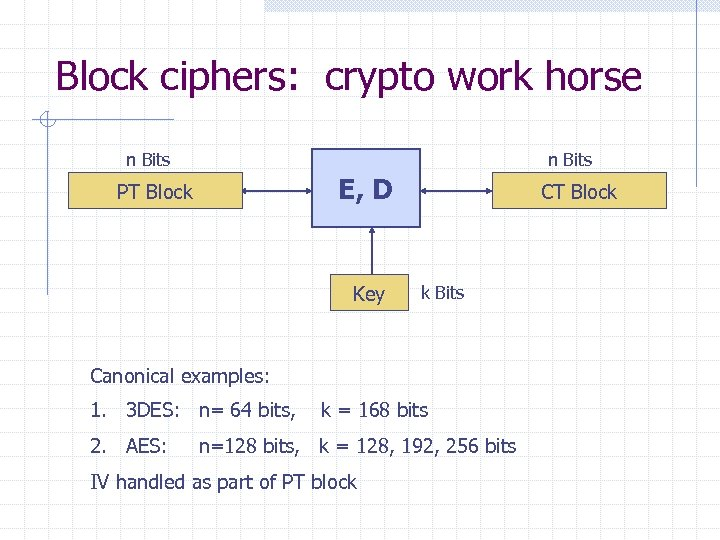Block ciphers: crypto work horse n Bits E, D PT Block Key CT Block k Bits Canonical examples: 1. 3 DES: n= 64 bits, 2. AES: k = 168 bits n=128 bits, k = 128, 192, 256 bits IV handled as part of PT block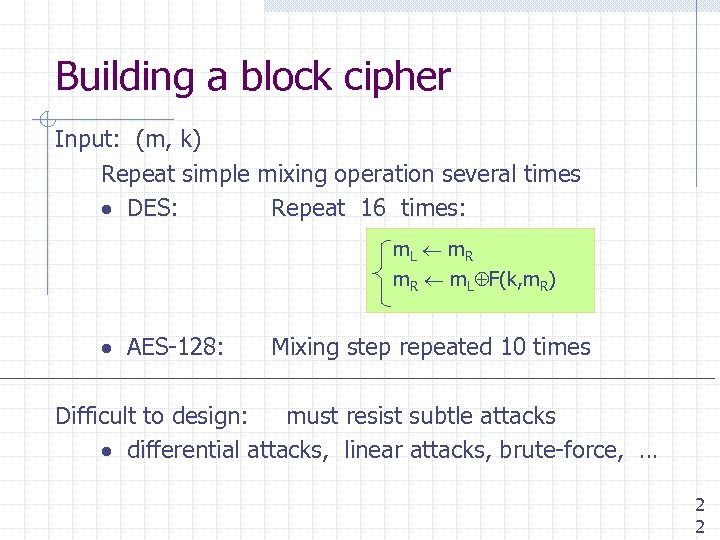Building a block cipher Input: (m, k) Repeat simple mixing operation several times DES: Repeat 16 times: m. L m R m. R m. L F(k, m. R) AES-128: Mixing step repeated 10 times Difficult to design: must resist subtle attacks differential attacks, linear attacks, brute-force, … 2 2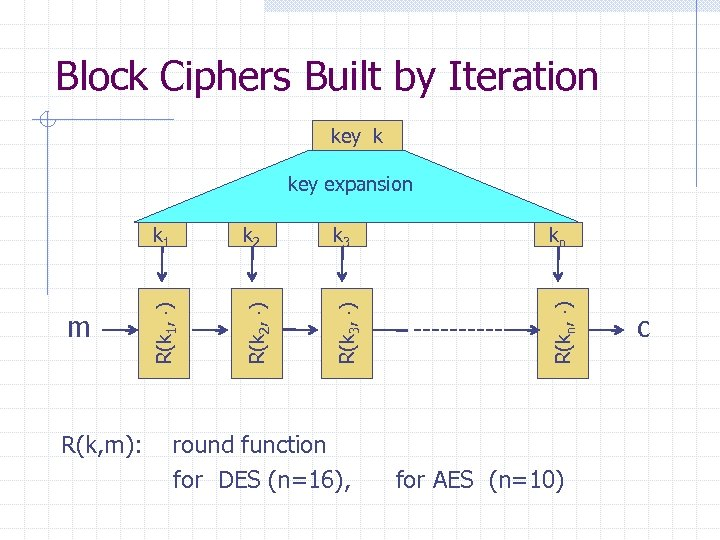Block Ciphers Built by Iteration key k k 1 R(k, m): k 3 kn R(k 2, ) R(k 3, ) R(kn, ) m k 2 R(k 1, ) key expansion round function for DES (n=16), for AES (n=10) c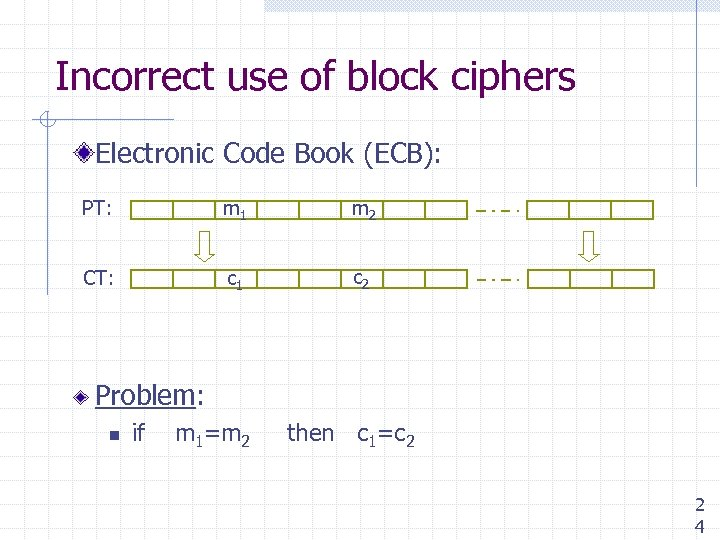Incorrect use of block ciphers Electronic Code Book (ECB): PT: m 1 m 2 CT: c 1 c 2 Problem: n if m 1=m 2 then c 1=c 2 2 4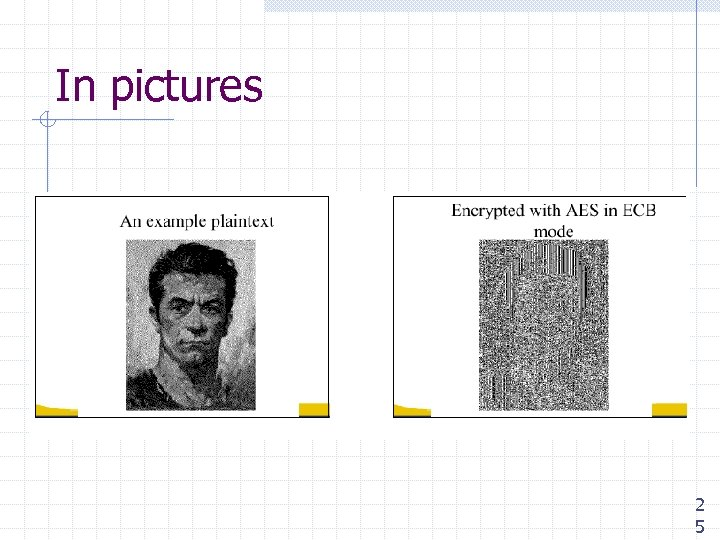In pictures 2 5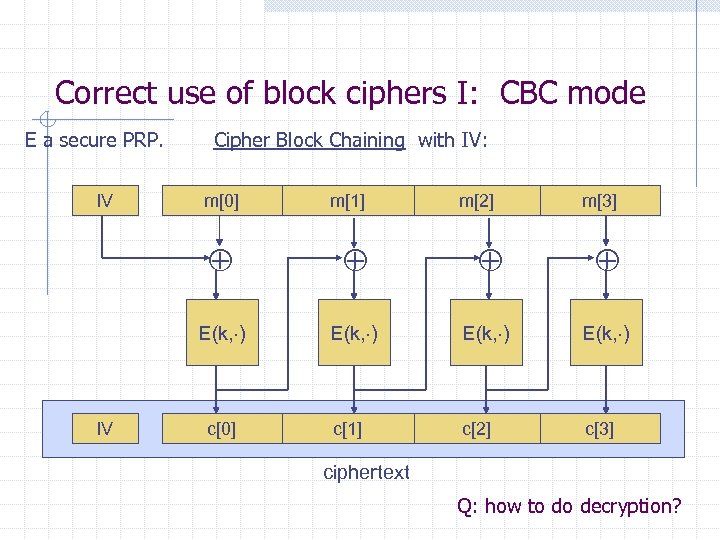Correct use of block ciphers I: CBC mode E a secure PRP. IV Cipher Block Chaining with IV: m m m m E(k, ) IV E(k, ) c c c c ciphertext Q: how to do decryption?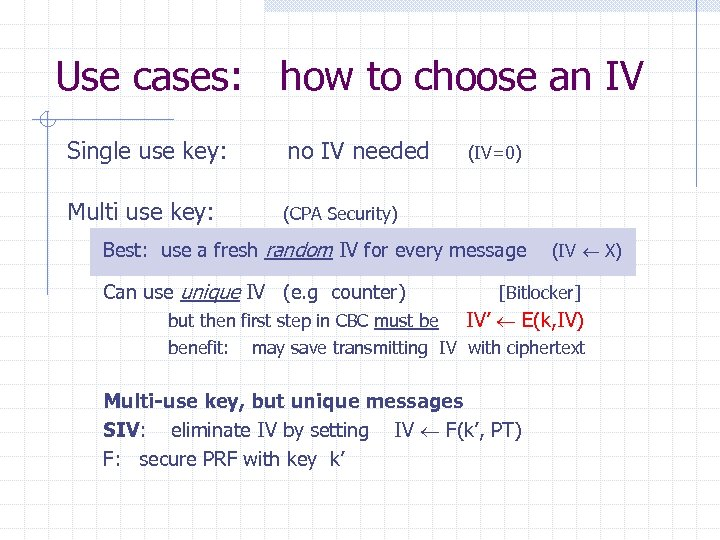Use cases: how to choose an IV Single use key: no IV needed Multi use key: (CPA Security) (IV=0) Best: use a fresh random IV for every message Can use unique IV (e. g counter) (IV X) [Bitlocker] but then first step in CBC must be IV’ E(k, IV) benefit: may save transmitting IV with ciphertext Multi-use key, but unique messages SIV: eliminate IV by setting IV F(k’, PT) F: secure PRF with key k’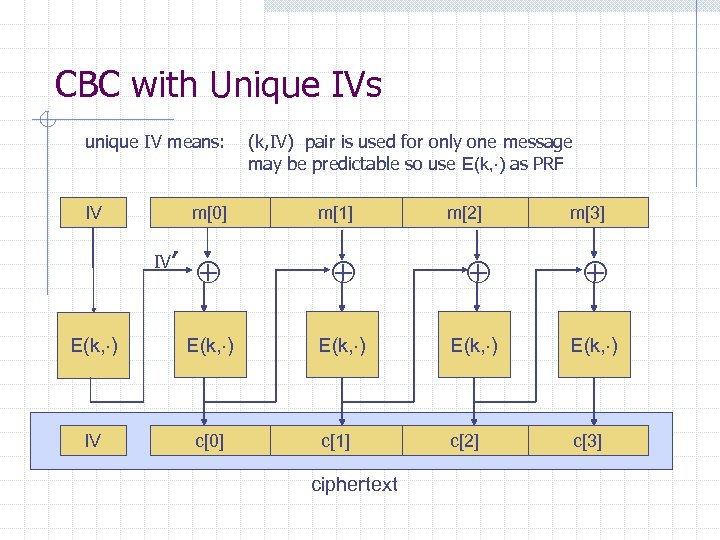CBC with Unique IVs unique IV means: IV m IV′ (k, IV) pair is used for only one message may be predictable so use E(k, ) as PRF m m m E(k, ) E(k, ) IV c c c c ciphertext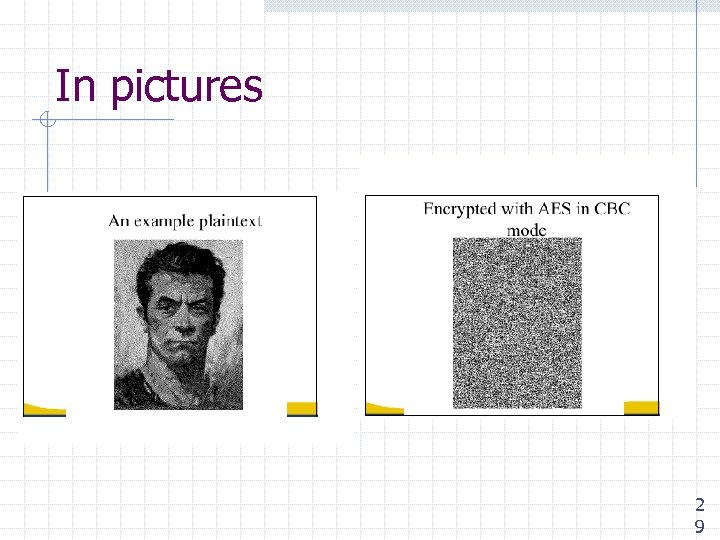In pictures 2 9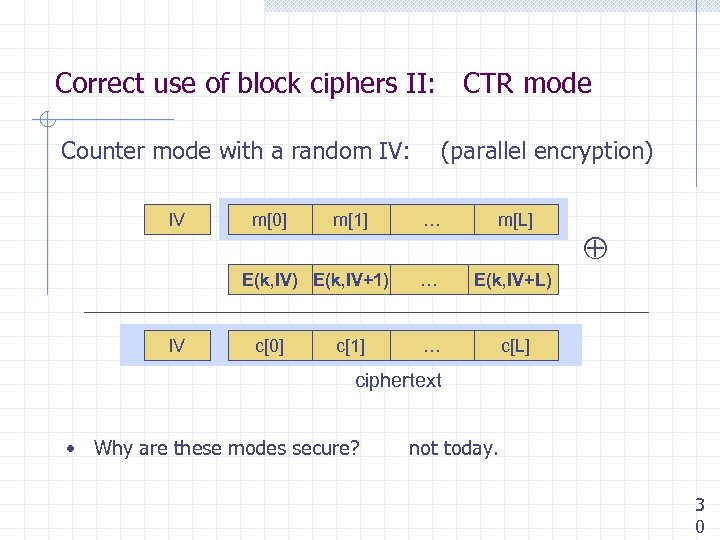Correct use of block ciphers II: CTR mode Counter mode with a random IV: IV m m E(k, IV) E(k, IV+1) IV c c (parallel encryption) … m[L] … E(k, IV+L) … c[L] ciphertext • Why are these modes secure? not today. 3 0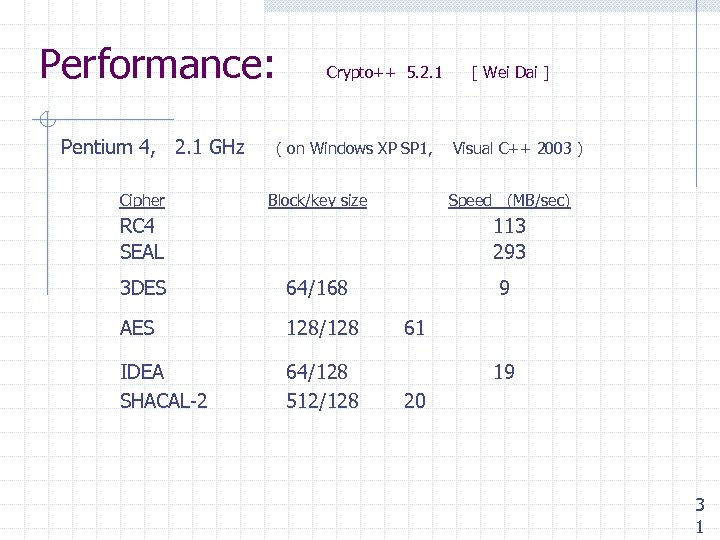Performance: Pentium 4, 2. 1 GHz Cipher Crypto++ 5. 2. 1 ( on Windows XP SP 1, Block/key size [ Wei Dai ] Visual C++ 2003 ) Speed (MB/sec) RC 4 SEAL 113 293 3 DES 64/168 AES 128/128 IDEA SHACAL-2 64/128 512/128 9 61 19 20 3 1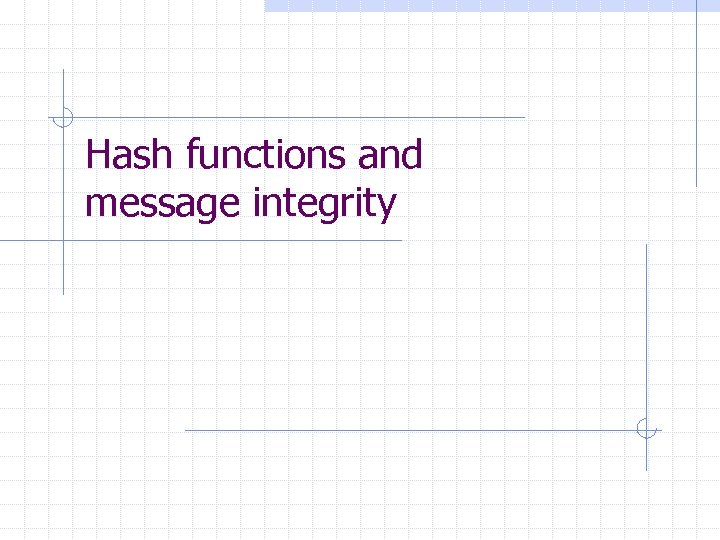Hash functions and message integrity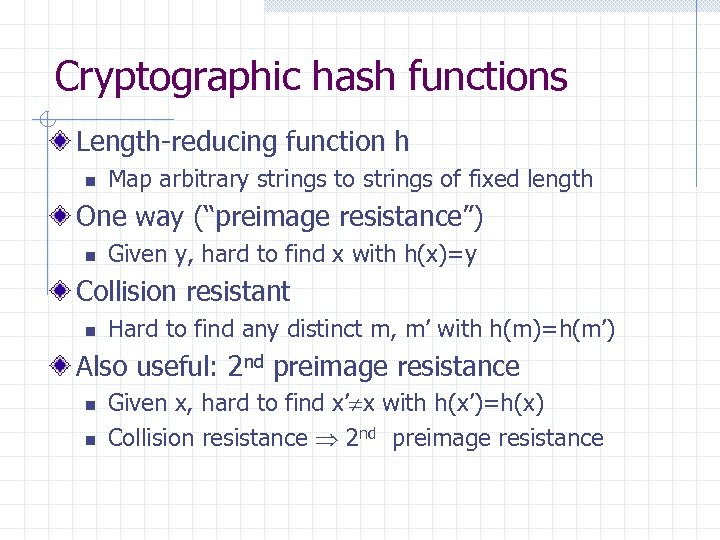Cryptographic hash functions Length-reducing function h n Map arbitrary strings to strings of fixed length One way (“preimage resistance”) n Given y, hard to find x with h(x)=y Collision resistant n Hard to find any distinct m, m’ with h(m)=h(m’) Also useful: 2 nd preimage resistance n n Given x, hard to find x’ x with h(x’)=h(x) Collision resistance 2 nd preimage resistance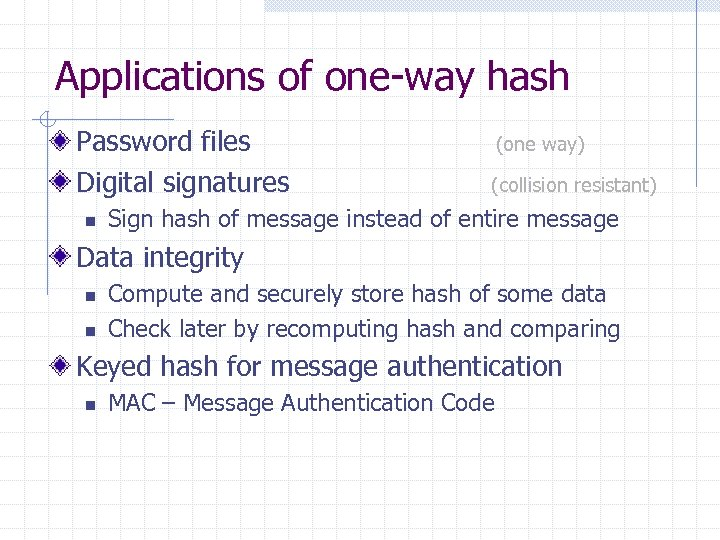Applications of one-way hash Password files Digital signatures n (one way) (collision resistant) Sign hash of message instead of entire message Data integrity n n Compute and securely store hash of some data Check later by recomputing hash and comparing Keyed hash for message authentication n MAC – Message Authentication Code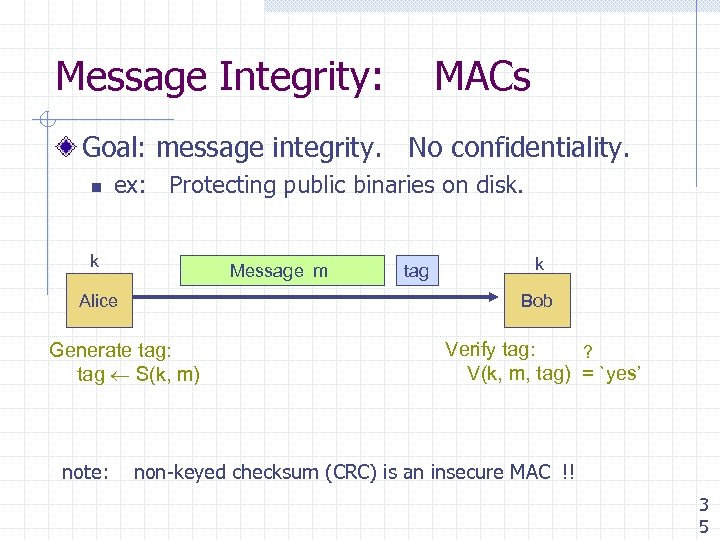Message Integrity: MACs Goal: message integrity. No confidentiality. n ex: Protecting public binaries on disk. k Message m Alice k Bob Generate tag: tag S(k, m) note: tag Verify tag: ? V(k, m, tag) = `yes’ non-keyed checksum (CRC) is an insecure MAC !! 3 5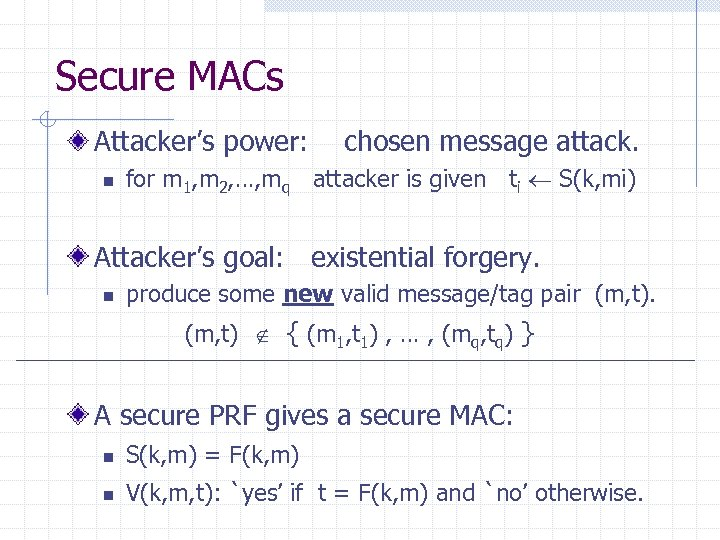Secure MACs Attacker’s power: n chosen message attack. for m 1, m 2, …, mq attacker is given ti S(k, mi) Attacker’s goal: existential forgery. n produce some new valid message/tag pair (m, t) { (m 1, t 1) , … , (mq, tq) } A secure PRF gives a secure MAC: n S(k, m) = F(k, m) n V(k, m, t): `yes’ if t = F(k, m) and `no’ otherwise.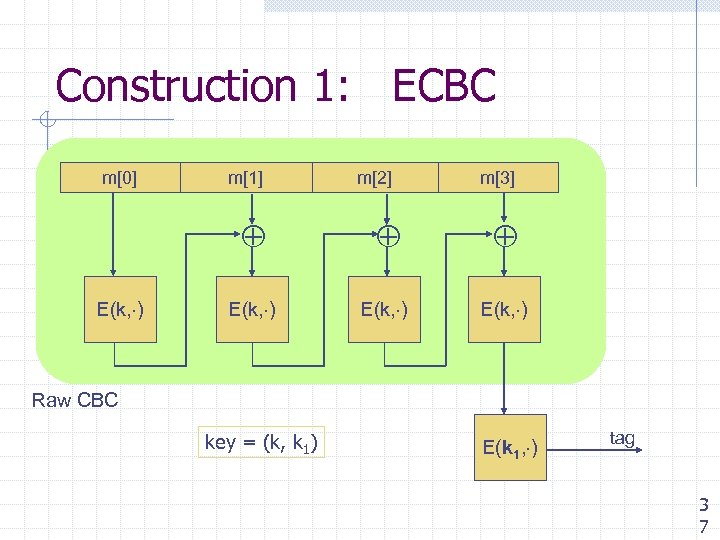Construction 1: ECBC m m m m E(k, ) Raw CBC key = (k, k 1) E(k 1, ) tag 3 7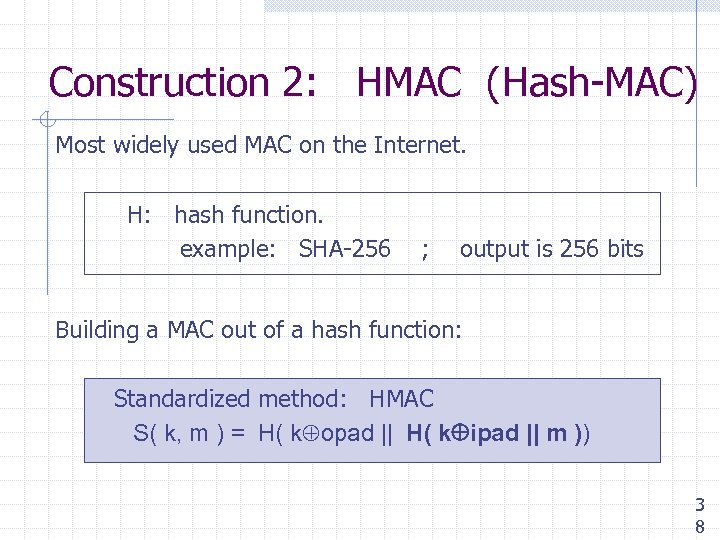Construction 2: HMAC (Hash-MAC) Most widely used MAC on the Internet. H: hash function. example: SHA-256 ; output is 256 bits Building a MAC out of a hash function: Standardized method: HMAC S( k, m ) = H( k opad || H( k ipad || m )) 3 8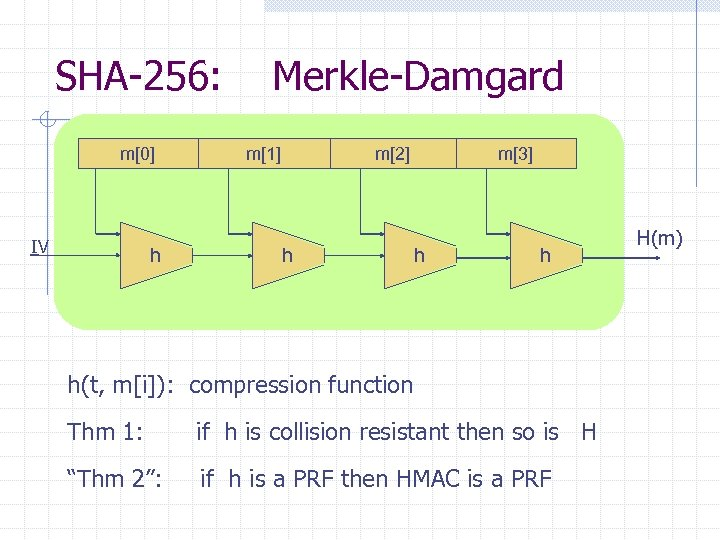SHA-256: m IV h Merkle-Damgard m m h m h h h(t, m[i]): compression function Thm 1: if h is collision resistant then so is H “Thm 2”: if h is a PRF then HMAC is a PRF H(m)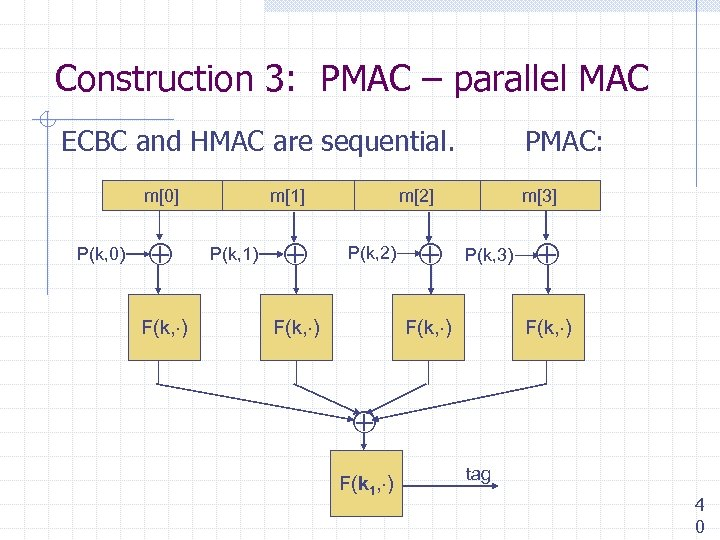Construction 3: PMAC – parallel MAC ECBC and HMAC are sequential. m P(k, 0) F(k, ) m P(k, 1) PMAC: m P(k, 2) F(k, ) m P(k, 3) F(k, ) F(k 1, ) tag 4 0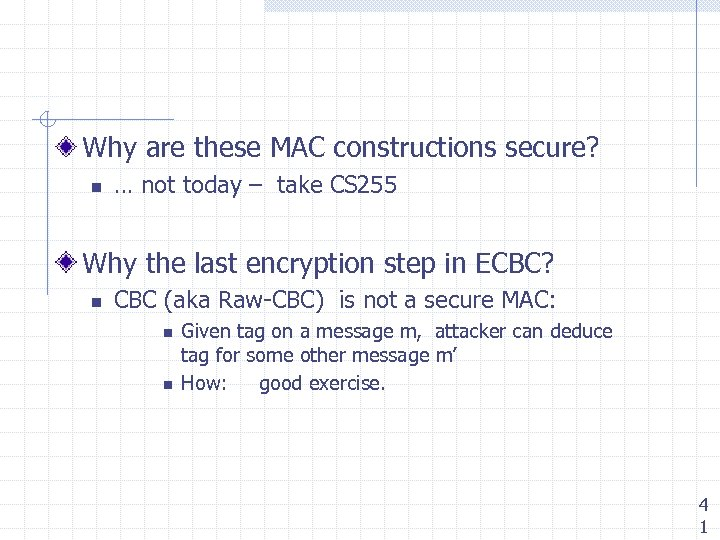Why are these MAC constructions secure? n … not today – take CS 255 Why the last encryption step in ECBC? n CBC (aka Raw-CBC) is not a secure MAC: n n Given tag on a message m, attacker can deduce tag for some other message m’ How: good exercise. 4 1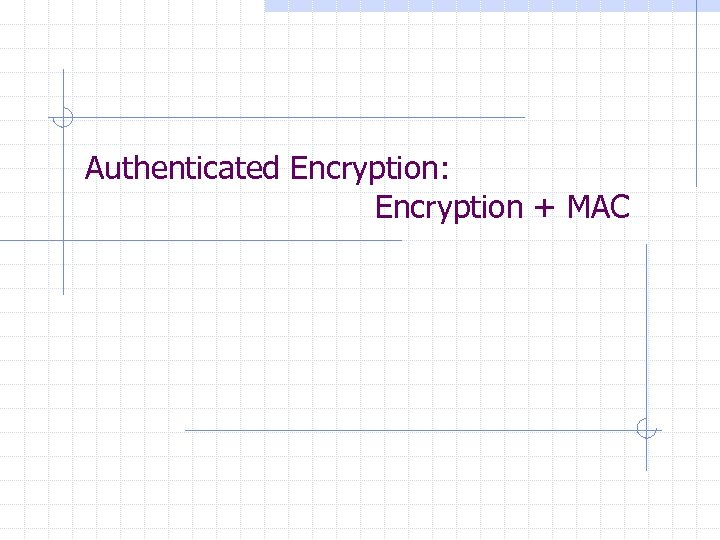Authenticated Encryption: Encryption + MAC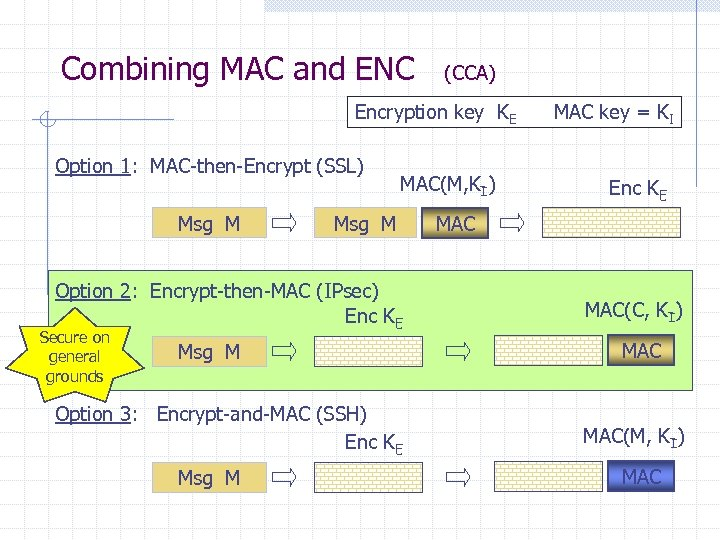Combining MAC and ENC (CCA) Encryption key KE Option 1: MAC-then-Encrypt (SSL) Msg M MAC(M, KI) Msg M Option 2: Encrypt-then-MAC (IPsec) Enc KE Secure on general grounds Msg M Option 3: Encrypt-and-MAC (SSH) Enc KE Msg M MAC key = KI Enc KE MAC(C, KI) MAC(M, KI) MAC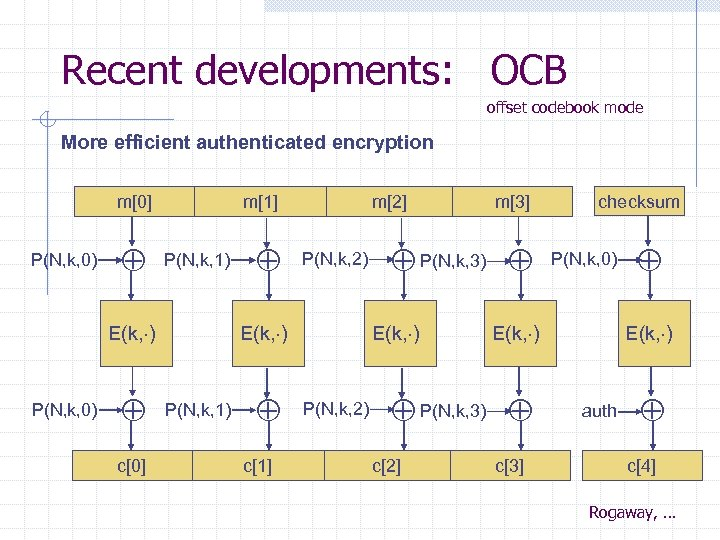Recent developments: OCB offset codebook mode More efficient authenticated encryption m P(N, k, 0) m P(N, k, 1) E(k, ) P(N, k, 0) c m P(N, k, 2) E(k, ) P(N, k, 1) c P(N, k, 3) E(k, ) P(N, k, 2) m P(N, k, 0) E(k, ) P(N, k, 3) c checksum c E(k, ) auth c Rogaway, …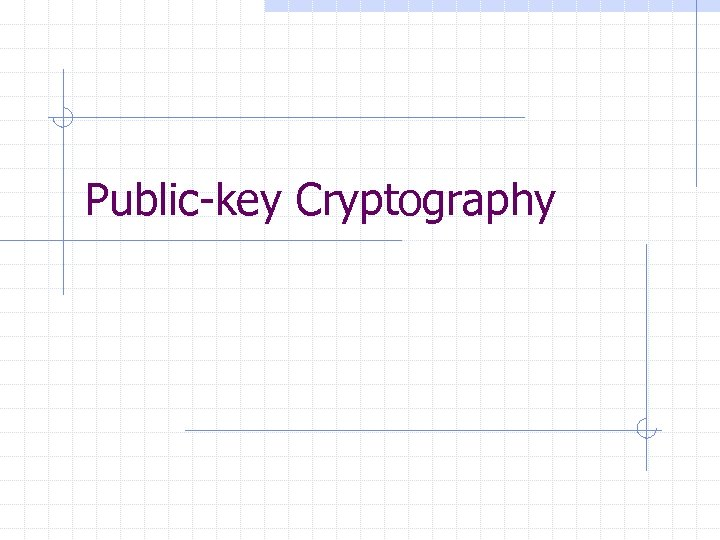Public-key Cryptography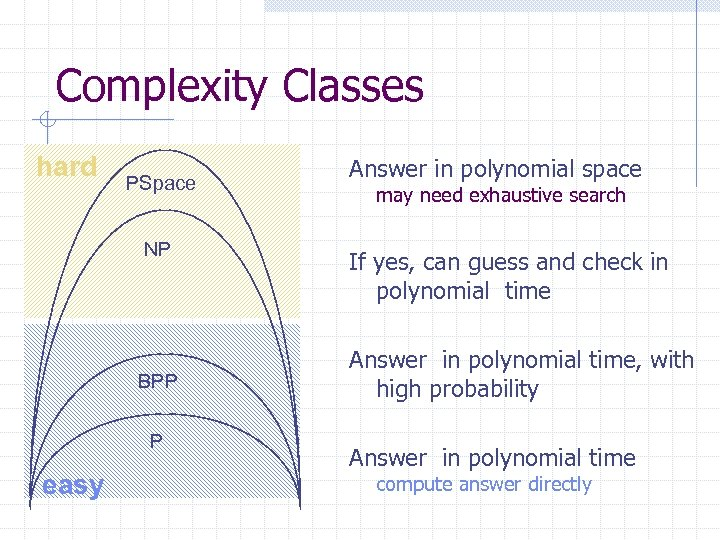Complexity Classes hard PSpace NP BPP P easy Answer in polynomial space may need exhaustive search If yes, can guess and check in polynomial time Answer in polynomial time, with high probability Answer in polynomial time compute answer directly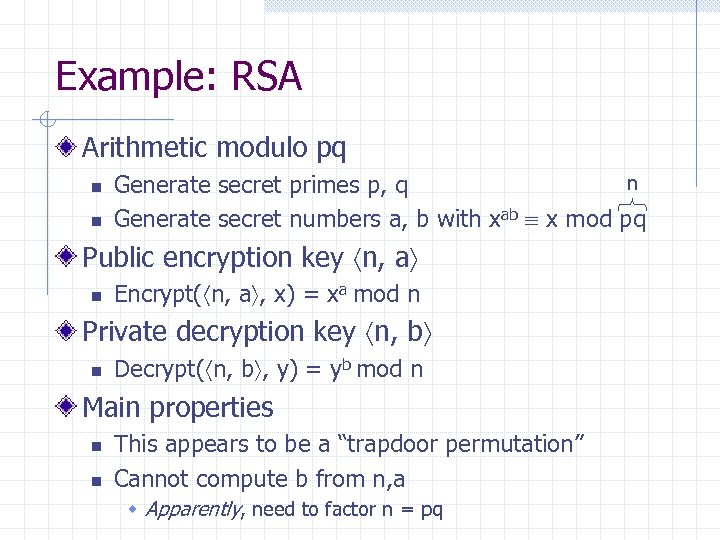Example: RSA Arithmetic modulo pq n n n Generate secret primes p, q Generate secret numbers a, b with xab x mod pq Public encryption key n, a n Encrypt( n, a , x) = xa mod n Private decryption key n, b n Decrypt( n, b , y) = yb mod n Main properties n n This appears to be a “trapdoor permutation” Cannot compute b from n, a w Apparently, need to factor n = pq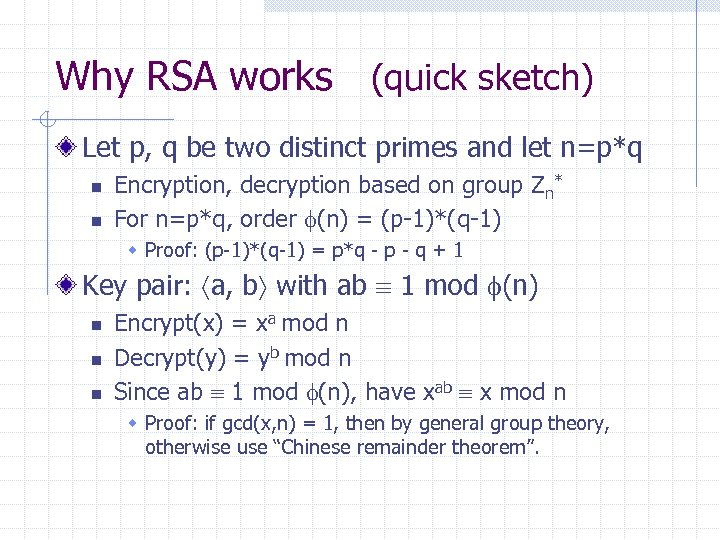Why RSA works (quick sketch) Let p, q be two distinct primes and let n=p*q n n Encryption, decryption based on group Zn* For n=p*q, order (n) = (p-1)*(q-1) w Proof: (p-1)*(q-1) = p*q - p - q + 1 Key pair: a, b with ab 1 mod (n) n n n Encrypt(x) = xa mod n Decrypt(y) = yb mod n Since ab 1 mod (n), have xab x mod n w Proof: if gcd(x, n) = 1, then by general group theory, otherwise use “Chinese remainder theorem”.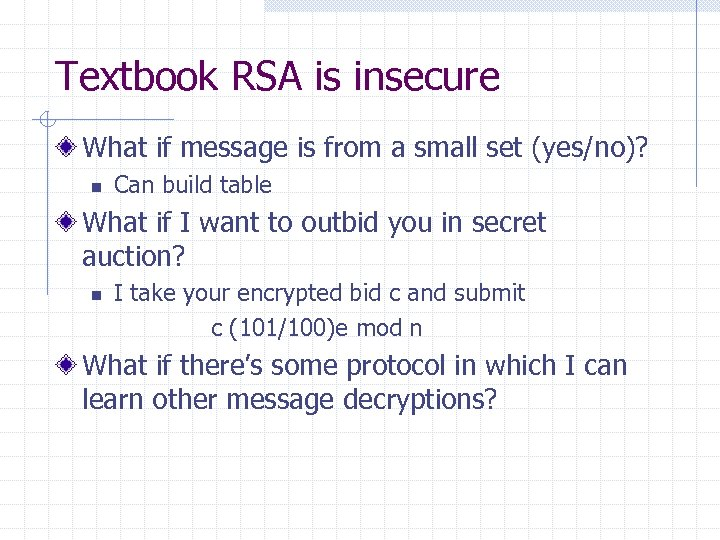Textbook RSA is insecure What if message is from a small set (yes/no)? n Can build table What if I want to outbid you in secret auction? n I take your encrypted bid c and submit c (101/100)e mod n What if there’s some protocol in which I can learn other message decryptions?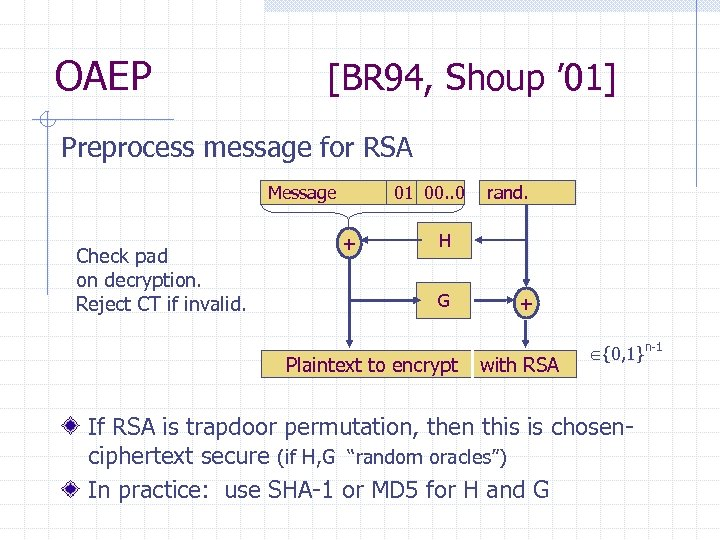OAEP [BR 94, Shoup ’ 01] Preprocess message for RSA Message Check pad on decryption. Reject CT if invalid. 01 00. . 0 + rand. H G Plaintext to encrypt + with RSA {0, 1}n-1 If RSA is trapdoor permutation, then this is chosenciphertext secure (if H, G “random oracles”) In practice: use SHA-1 or MD 5 for H and G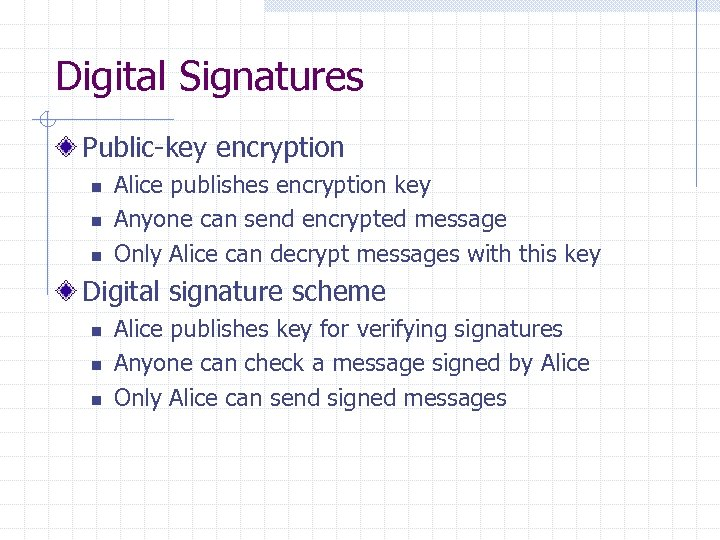Digital Signatures Public-key encryption n Alice publishes encryption key Anyone can send encrypted message Only Alice can decrypt messages with this key Digital signature scheme n n n Alice publishes key for verifying signatures Anyone can check a message signed by Alice Only Alice can send signed messages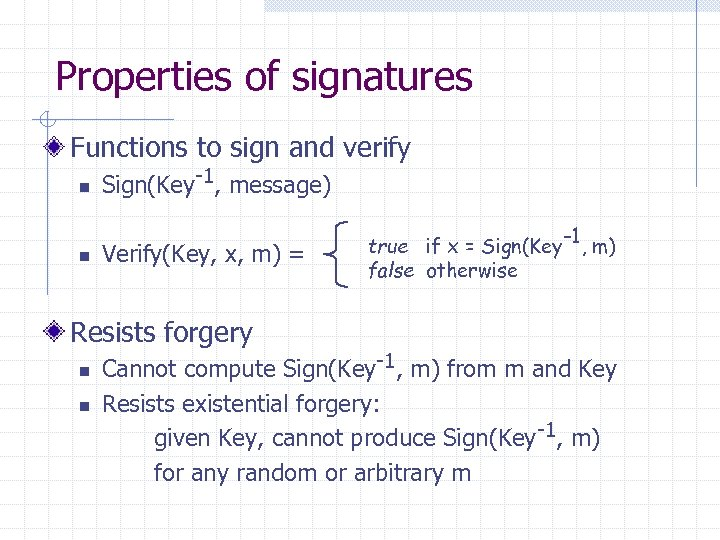Properties of signatures Functions to sign and verify n n Sign(Key-1, message) Verify(Key, x, m) = Resists forgery n n true if x = Sign(Key-1, m) false otherwise Cannot compute Sign(Key-1, m) from m and Key Resists existential forgery: given Key, cannot produce Sign(Key-1, m) for any random or arbitrary m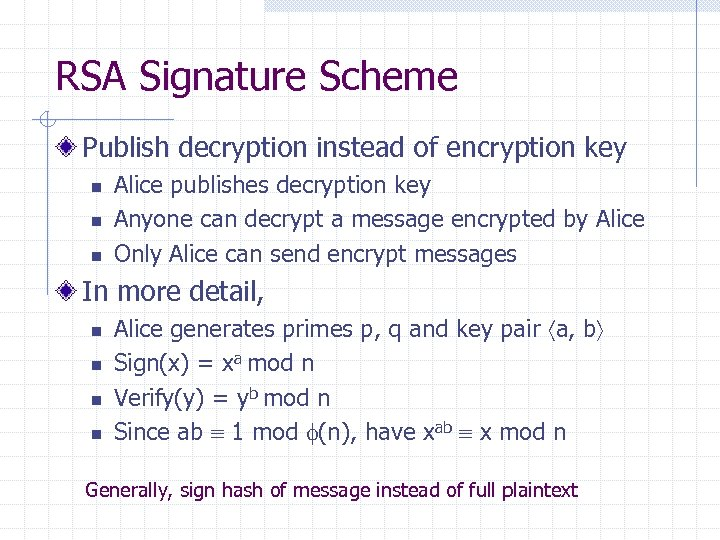RSA Signature Scheme Publish decryption instead of encryption key n n n Alice publishes decryption key Anyone can decrypt a message encrypted by Alice Only Alice can send encrypt messages In more detail, n n Alice generates primes p, q and key pair a, b Sign(x) = xa mod n Verify(y) = yb mod n Since ab 1 mod (n), have xab x mod n Generally, sign hash of message instead of full plaintext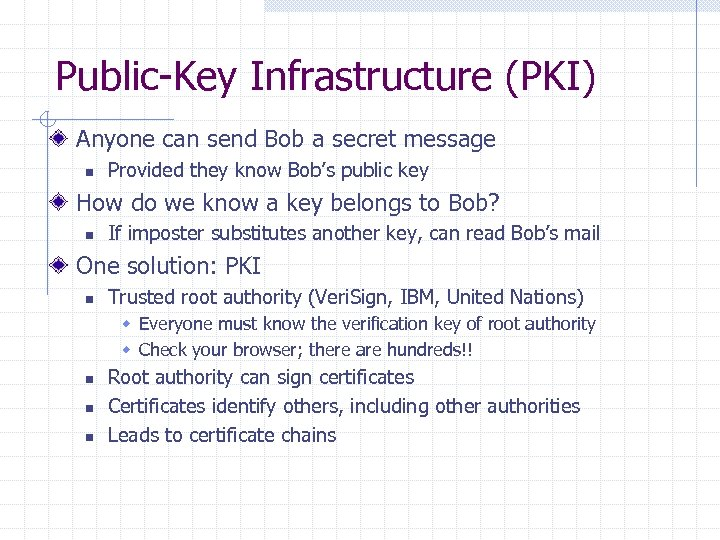Public-Key Infrastructure (PKI) Anyone can send Bob a secret message n Provided they know Bob’s public key How do we know a key belongs to Bob? n If imposter substitutes another key, can read Bob’s mail One solution: PKI n Trusted root authority (Veri. Sign, IBM, United Nations) w Everyone must know the verification key of root authority w Check your browser; there are hundreds!! n n n Root authority can sign certificates Certificates identify others, including other authorities Leads to certificate chains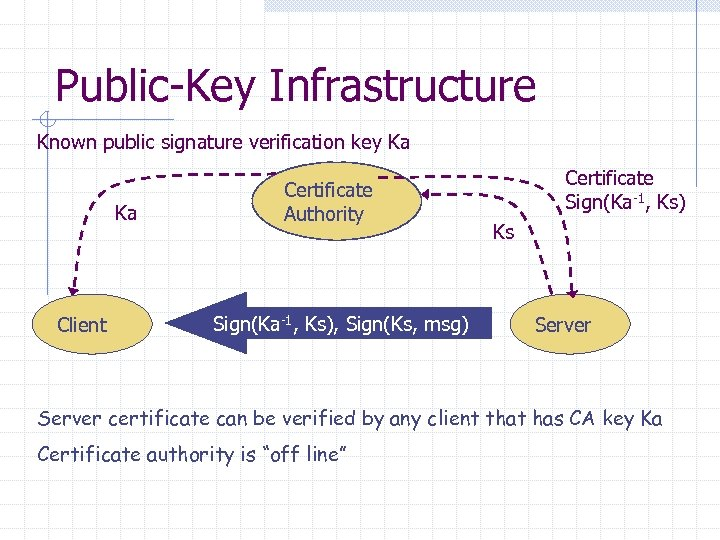Public-Key Infrastructure Known public signature verification key Ka Ka Client Certificate Authority Sign(Ka-1, Ks), Sign(Ks, msg) Certificate Sign(Ka-1, Ks) Ks Server certificate can be verified by any client that has CA key Ka Certificate authority is “off line”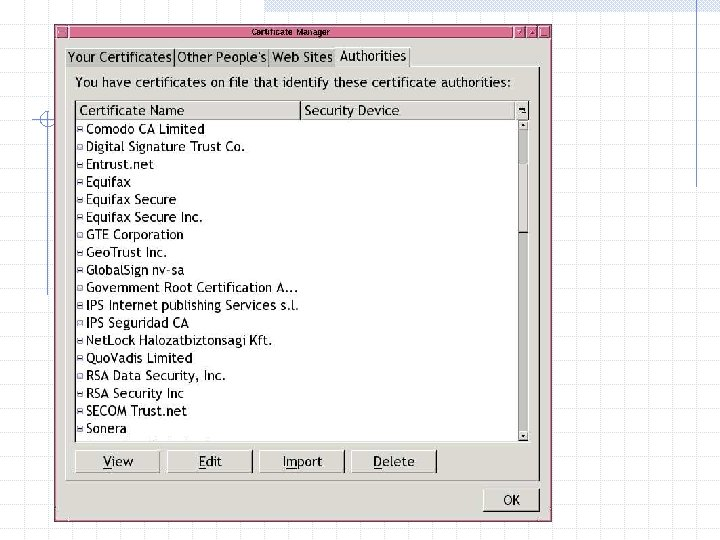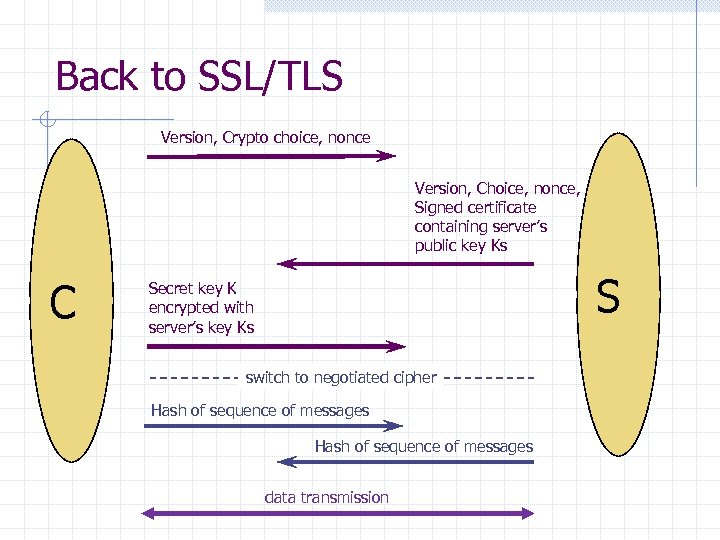Back to SSL/TLS Version, Crypto choice, nonce Version, Choice, nonce, Signed certificate containing server’s public key Ks C S Secret key K encrypted with server’s key Ks switch to negotiated cipher Hash of sequence of messages data transmission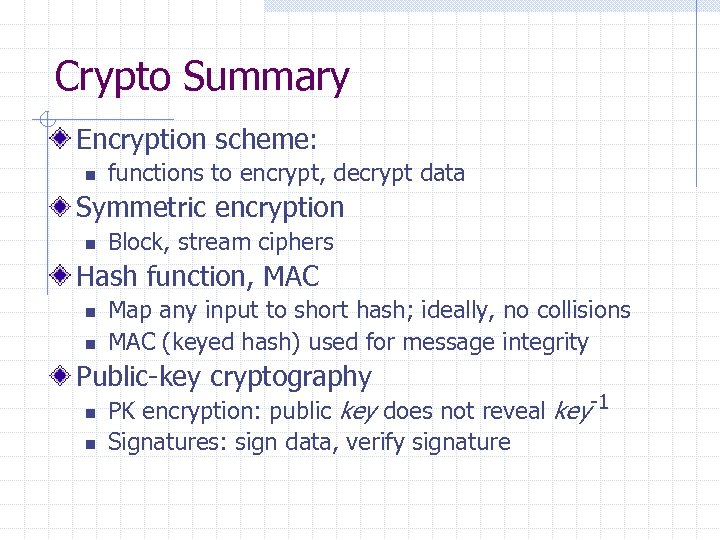Crypto Summary Encryption scheme: n functions to encrypt, decrypt data Symmetric encryption n Block, stream ciphers Hash function, MAC n n Map any input to short hash; ideally, no collisions MAC (keyed hash) used for message integrity Public-key cryptography n n PK encryption: public key does not reveal key-1 Signatures: sign data, verify signature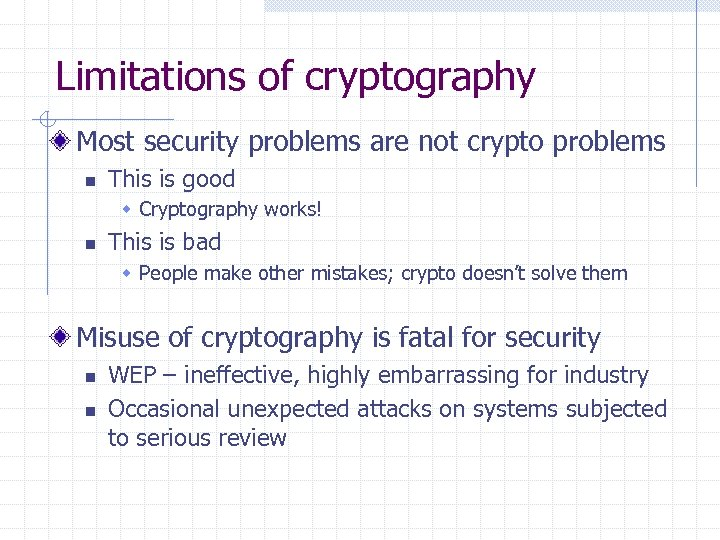Limitations of cryptography Most security problems are not crypto problems n This is good w Cryptography works! n This is bad w People make other mistakes; crypto doesn’t solve them Misuse of cryptography is fatal for security n n WEP – ineffective, highly embarrassing for industry Occasional unexpected attacks on systems subjected to serious review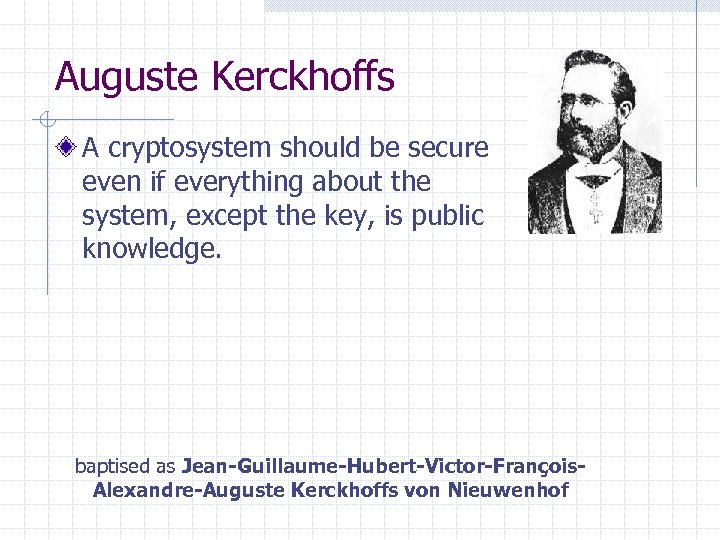Auguste Kerckhoffs A cryptosystem should be secure even if everything about the system, except the key, is public knowledge. baptised as Jean-Guillaume-Hubert-Victor-François. Alexandre-Auguste Kerckhoffs von Nieuwenhof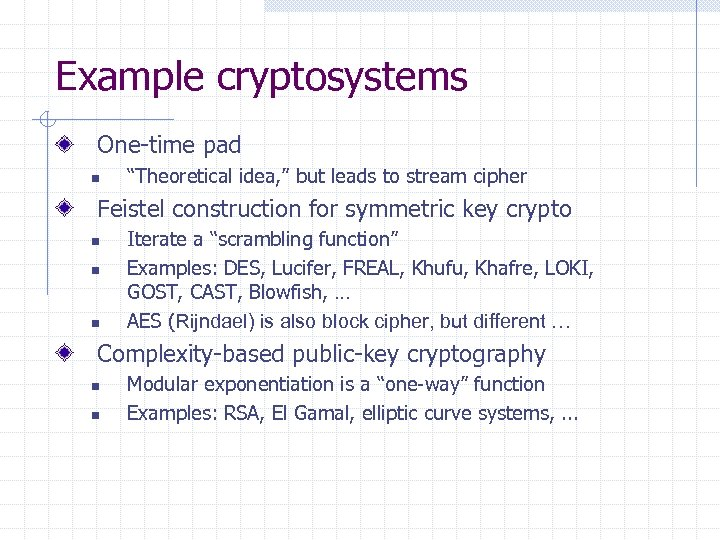Example cryptosystems One-time pad n “Theoretical idea, ” but leads to stream cipher Feistel construction for symmetric key crypto n n n Iterate a “scrambling function” Examples: DES, Lucifer, FREAL, Khufu, Khafre, LOKI, GOST, CAST, Blowfish, … AES (Rijndael) is also block cipher, but different … Complexity-based public-key cryptography n n Modular exponentiation is a “one-way” function Examples: RSA, El Gamal, elliptic curve systems, . . .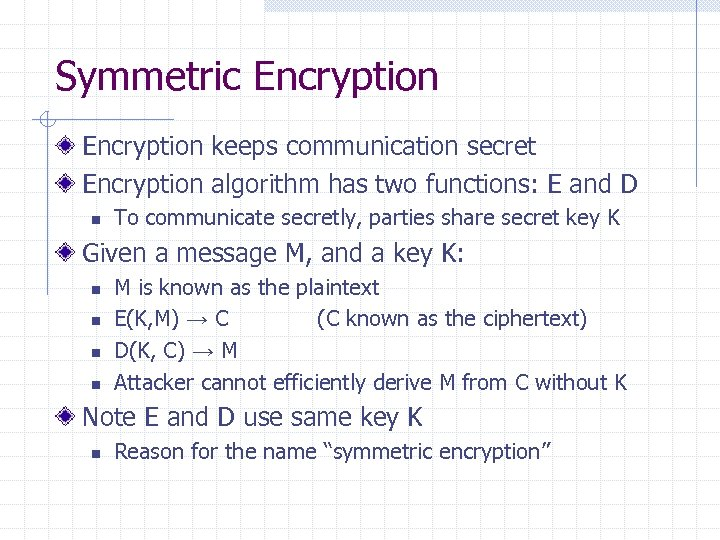Symmetric Encryption keeps communication secret Encryption algorithm has two functions: E and D n To communicate secretly, parties share secret key K Given a message M, and a key K: n n M is known as the plaintext E(K, M) → C (C known as the ciphertext) D(K, C) → M Attacker cannot efficiently derive M from C without K Note E and D use same key K n Reason for the name “symmetric encryption”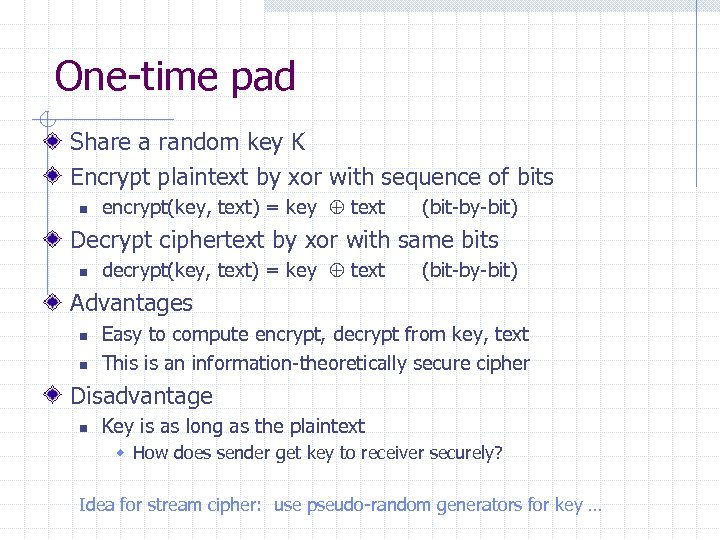One-time pad Share a random key K Encrypt plaintext by xor with sequence of bits n encrypt(key, text) = key text (bit-by-bit) Decrypt ciphertext by xor with same bits n decrypt(key, text) = key text (bit-by-bit) Advantages n n Easy to compute encrypt, decrypt from key, text This is an information-theoretically secure cipher Disadvantage n Key is as long as the plaintext w How does sender get key to receiver securely? Idea for stream cipher: use pseudo-random generators for key …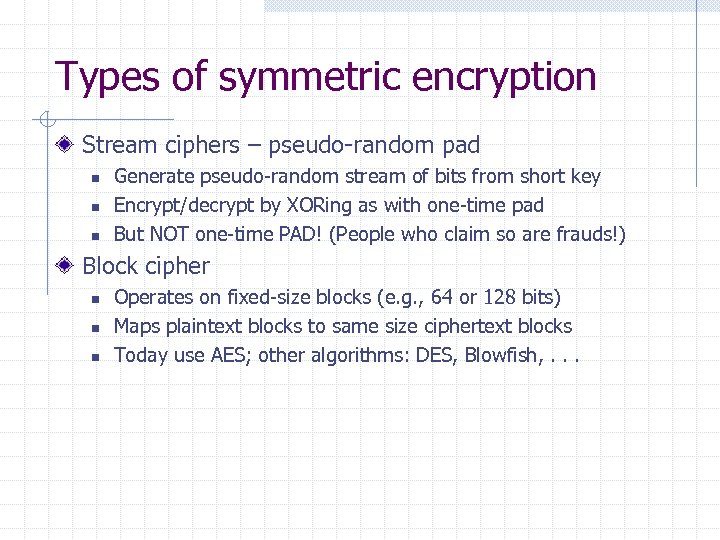Types of symmetric encryption Stream ciphers – pseudo-random pad n n n Generate pseudo-random stream of bits from short key Encrypt/decrypt by XORing as with one-time pad But NOT one-time PAD! (People who claim so are frauds!) Block cipher n n n Operates on fixed-size blocks (e. g. , 64 or 128 bits) Maps plaintext blocks to same size ciphertext blocks Today use AES; other algorithms: DES, Blowfish, . . .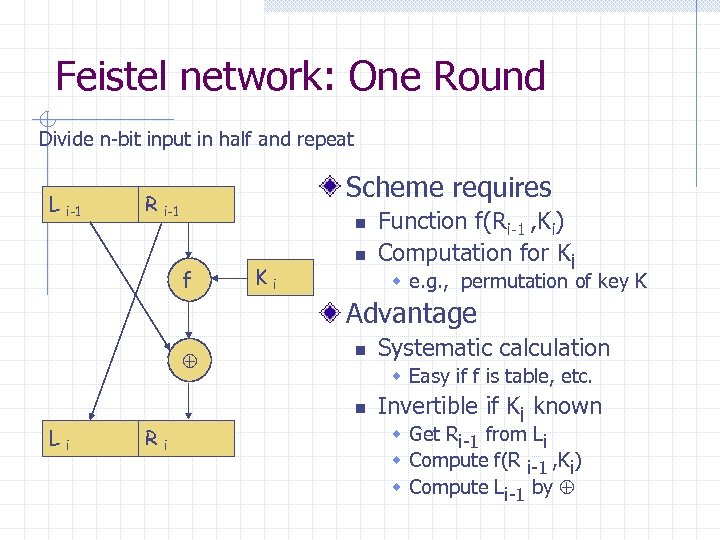Feistel network: One Round Divide n-bit input in half and repeat L i-1 Scheme requires R i-1 n f Ki n Function f(Ri-1 , Ki) Computation for Ki w e. g. , permutation of key K Advantage n w Easy if f is table, etc. n Li Ri Systematic calculation Invertible if Ki known w Get Ri-1 from Li w Compute f(R i-1 , Ki) w Compute Li-1 by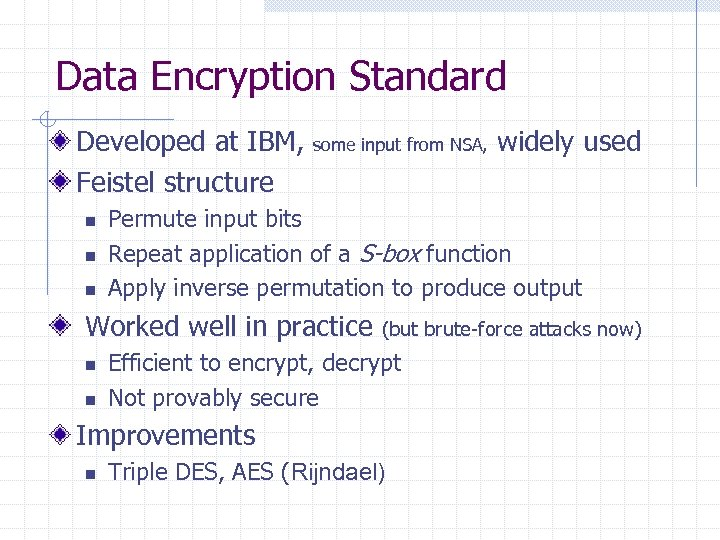Data Encryption Standard Developed at IBM, Feistel structure n n n some input from NSA, Permute input bits Repeat application of a S-box function Apply inverse permutation to produce output Worked well in practice n n (but brute-force attacks now) Efficient to encrypt, decrypt Not provably secure Improvements n widely used Triple DES, AES (Rijndael)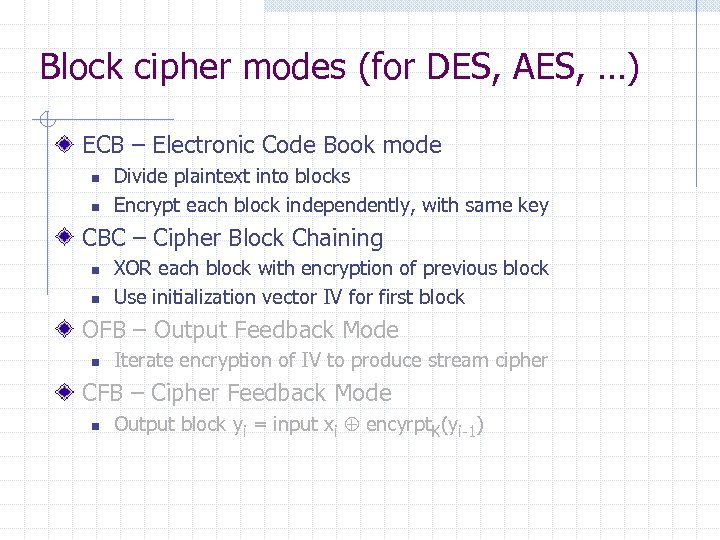Block cipher modes (for DES, AES, …) ECB – Electronic Code Book mode n n Divide plaintext into blocks Encrypt each block independently, with same key CBC – Cipher Block Chaining n n XOR each block with encryption of previous block Use initialization vector IV for first block OFB – Output Feedback Mode n Iterate encryption of IV to produce stream cipher CFB – Cipher Feedback Mode n Output block yi = input xi encyrpt. K(yi-1)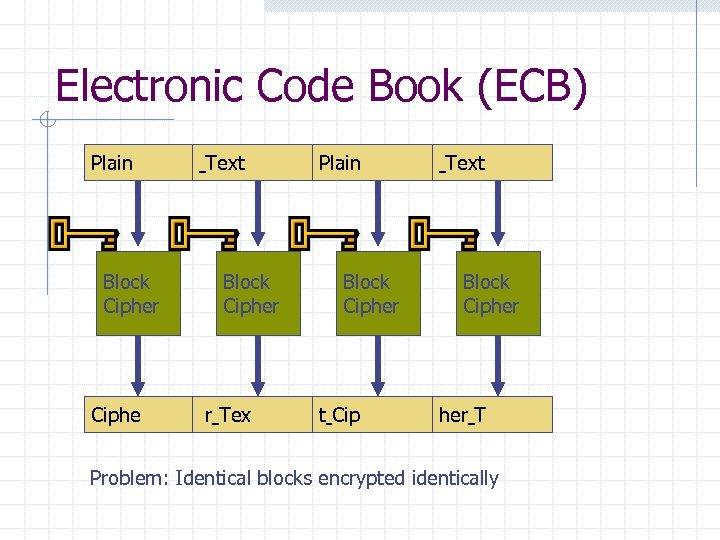Electronic Code Book (ECB) Plain Block Cipher Ciphe Text Block Cipher r Tex Plain Block Cipher t Cip Text Block Cipher T Problem: Identical blocks encrypted identically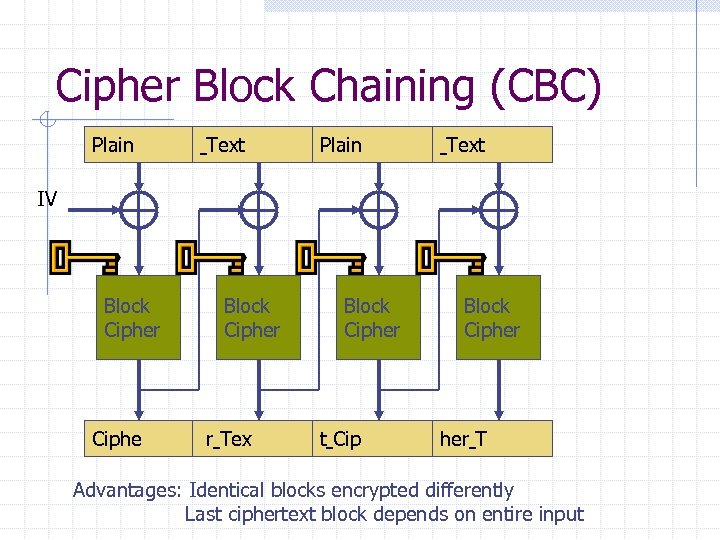Cipher Block Chaining (CBC) Plain Text IV Block Cipher Ciphe Block Cipher r Tex Block Cipher t Cip Block Cipher T Advantages: Identical blocks encrypted differently Last ciphertext block depends on entire input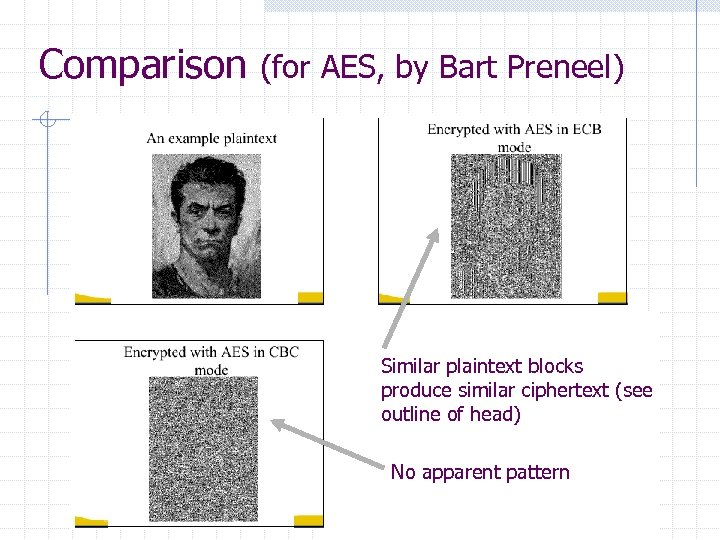Comparison (for AES, by Bart Preneel) Similar plaintext blocks produce similar ciphertext (see outline of head) No apparent pattern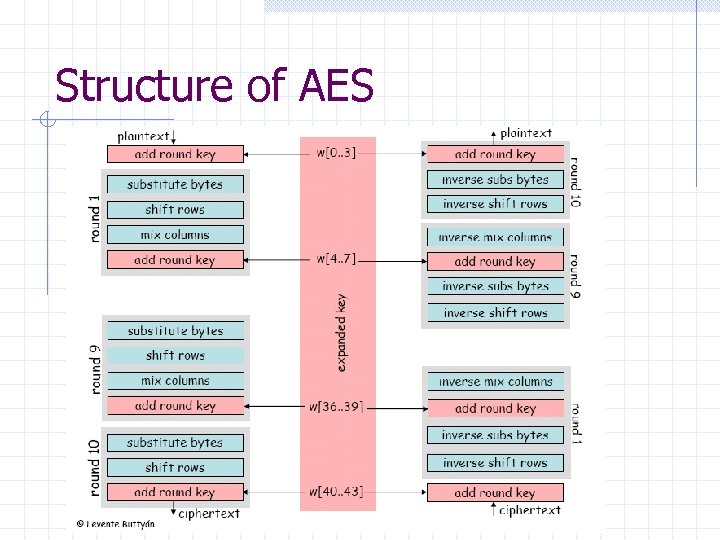Structure of AES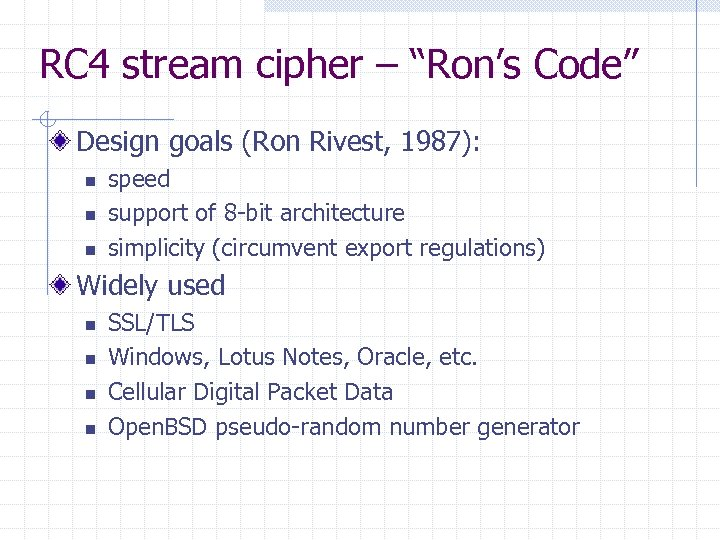RC 4 stream cipher – “Ron’s Code” Design goals (Ron Rivest, 1987): n n n speed support of 8 -bit architecture simplicity (circumvent export regulations) Widely used n n SSL/TLS Windows, Lotus Notes, Oracle, etc. Cellular Digital Packet Data Open. BSD pseudo-random number generator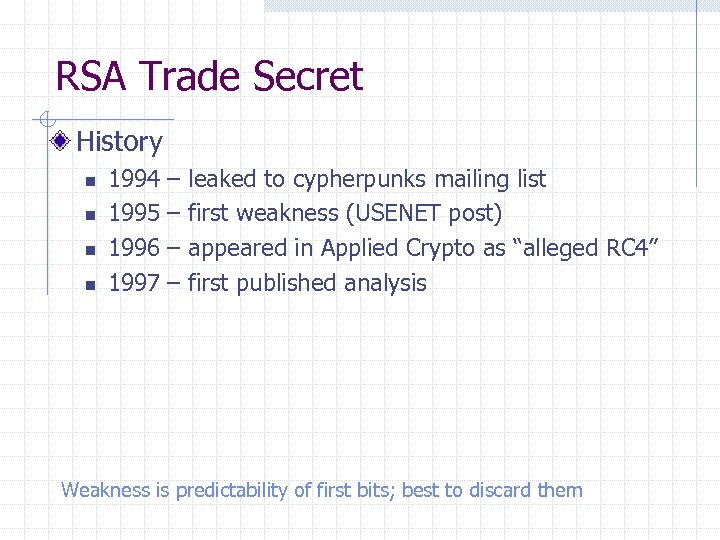RSA Trade Secret History n n 1994 1995 1996 1997 – – leaked to cypherpunks mailing list first weakness (USENET post) appeared in Applied Crypto as “alleged RC 4” first published analysis Weakness is predictability of first bits; best to discard them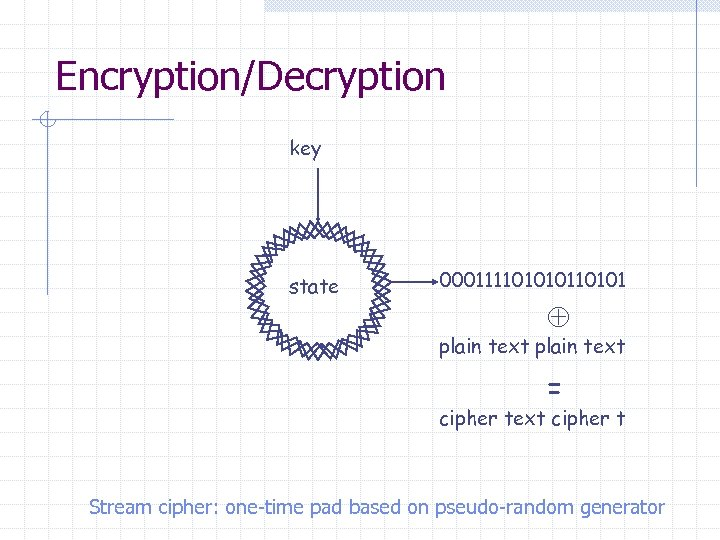Encryption/Decryption key state 0001111010101 plain text = cipher text cipher t Stream cipher: one-time pad based on pseudo-random generator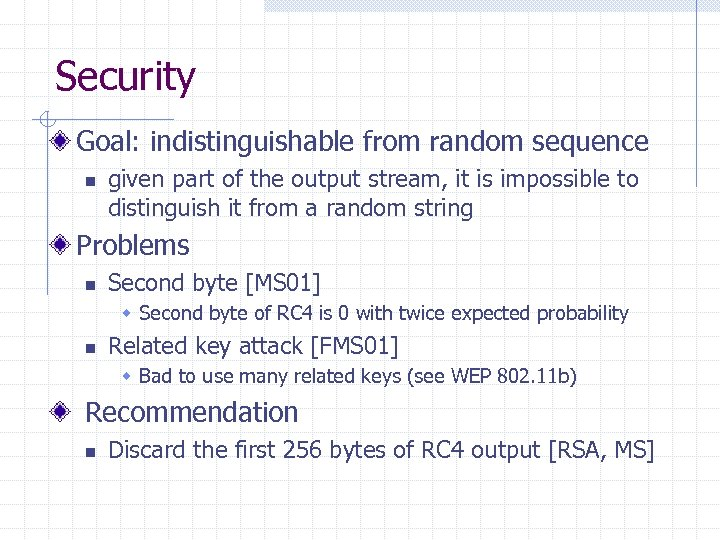Security Goal: indistinguishable from random sequence n given part of the output stream, it is impossible to distinguish it from a random string Problems n Second byte [MS 01] w Second byte of RC 4 is 0 with twice expected probability n Related key attack [FMS 01] w Bad to use many related keys (see WEP 802. 11 b) Recommendation n Discard the first 256 bytes of RC 4 output [RSA, MS]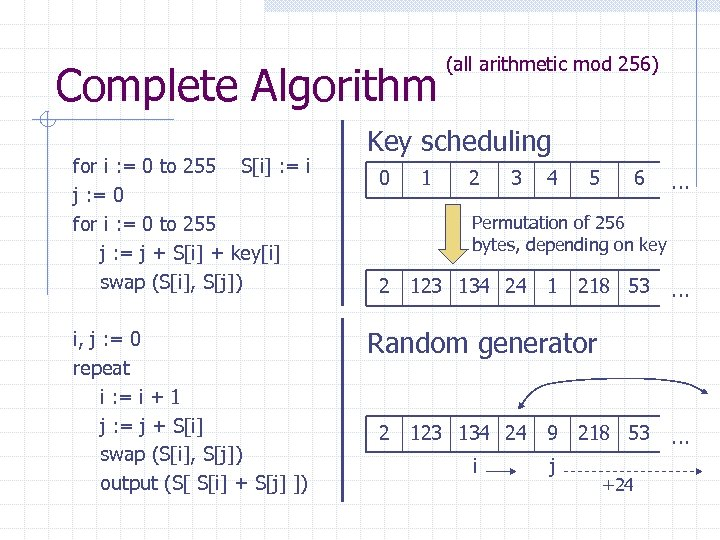Complete Algorithm for i : = 0 to 255 S[i] : = i j : = 0 for i : = 0 to 255 j : = j + S[i] + key[i] swap (S[i], S[j]) i, j : = 0 repeat i : = i + 1 j : = j + S[i] swap (S[i], S[j]) output (S[ S[i] + S[j] ]) (all arithmetic mod 256) Key scheduling 0 1 2 3 4 5 6 … Permutation of 256 bytes, depending on key 2 123 134 24 1 218 53 … Random generator 2 123 134 24 9 218 53 i j +24 …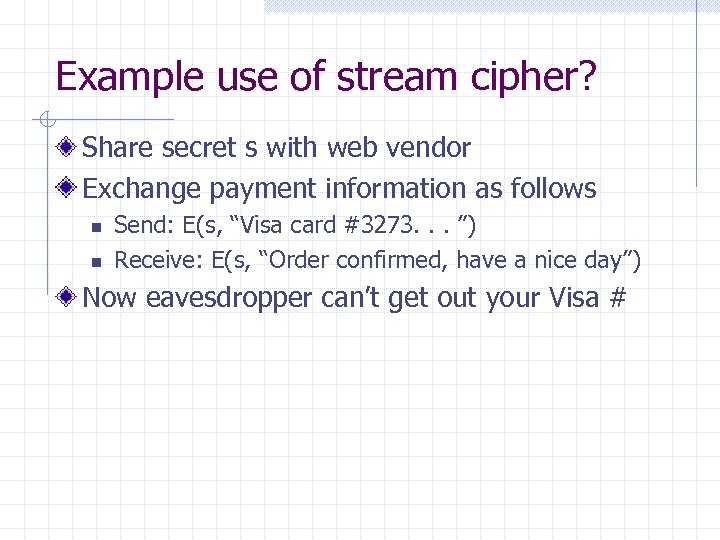Example use of stream cipher? Share secret s with web vendor Exchange payment information as follows n n Send: E(s, “Visa card #3273. . . ”) Receive: E(s, “Order confirmed, have a nice day”) Now eavesdropper can’t get out your Visa #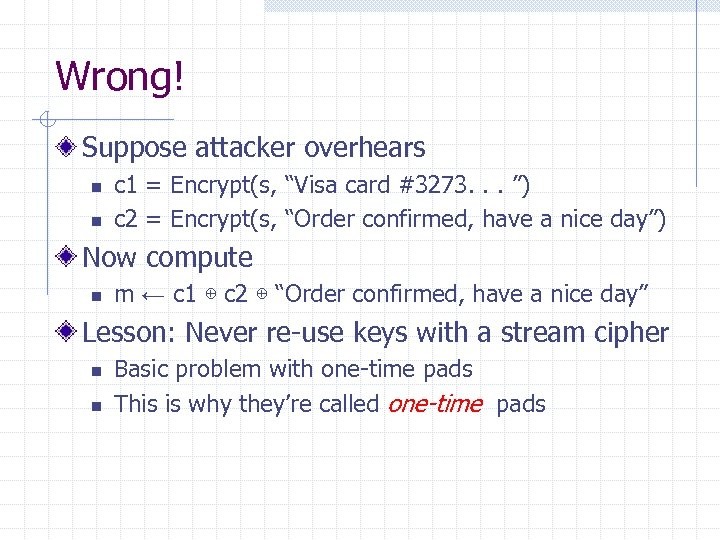Wrong! Suppose attacker overhears n n c 1 = Encrypt(s, “Visa card #3273. . . ”) c 2 = Encrypt(s, “Order confirmed, have a nice day”) Now compute n m ← c 1 ⊕ c 2 ⊕ “Order confirmed, have a nice day” Lesson: Never re-use keys with a stream cipher n n Basic problem with one-time pads This is why they’re called one-time pads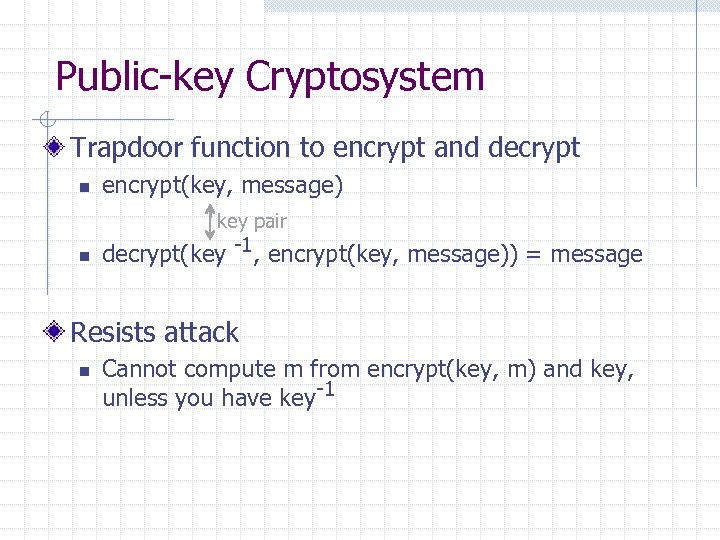Public-key Cryptosystem Trapdoor function to encrypt and decrypt n encrypt(key, message) key pair n decrypt(key -1, encrypt(key, message)) = message Resists attack n Cannot compute m from encrypt(key, m) and key, unless you have key-1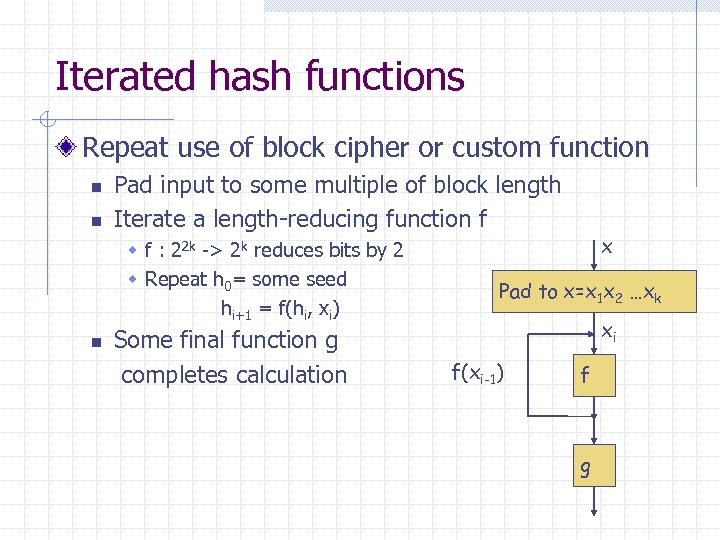Iterated hash functions Repeat use of block cipher or custom function n n Pad input to some multiple of block length Iterate a length-reducing function f w f : 22 k -> 2 k reduces bits by 2 w Repeat h 0= some seed hi+1 = f(hi, xi) n Some final function g completes calculation x Pad to x=x 1 x 2 …xk xi f(xi-1) f g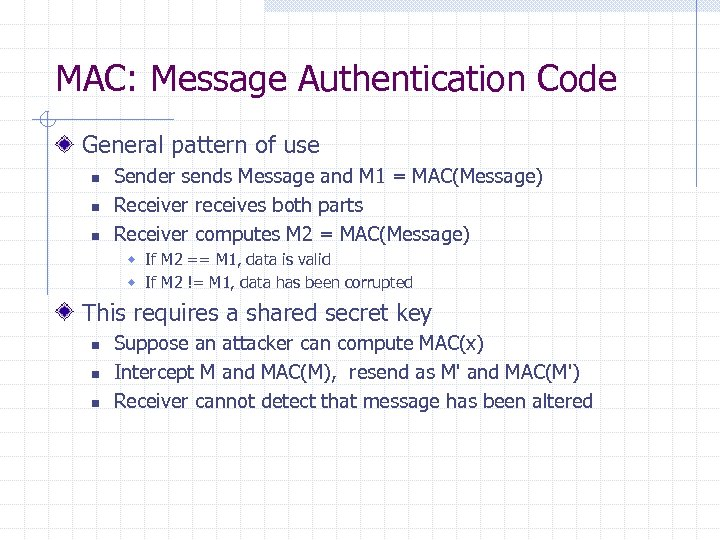MAC: Message Authentication Code General pattern of use n n n Sender sends Message and M 1 = MAC(Message) Receiver receives both parts Receiver computes M 2 = MAC(Message) w If M 2 == M 1, data is valid w If M 2 != M 1, data has been corrupted This requires a shared secret key n n n Suppose an attacker can compute MAC(x) Intercept M and MAC(M), resend as M' and MAC(M') Receiver cannot detect that message has been altered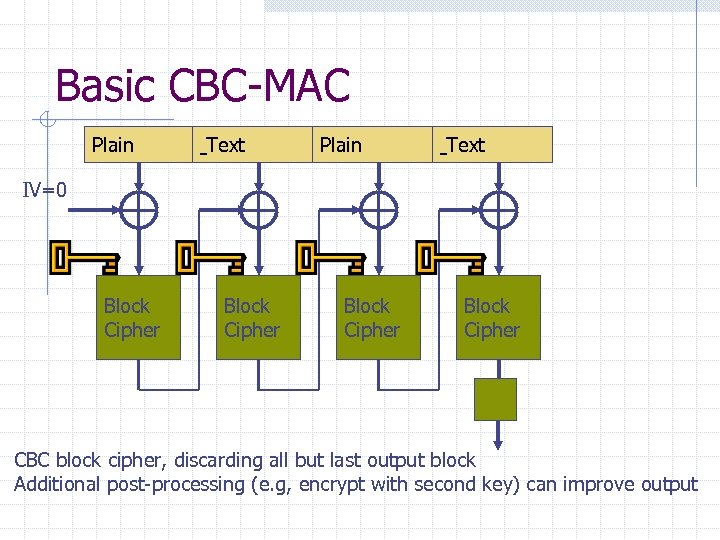Basic CBC-MAC Plain Text IV=0 Block Cipher CBC block cipher, discarding all but last output block Additional post-processing (e. g, encrypt with second key) can improve output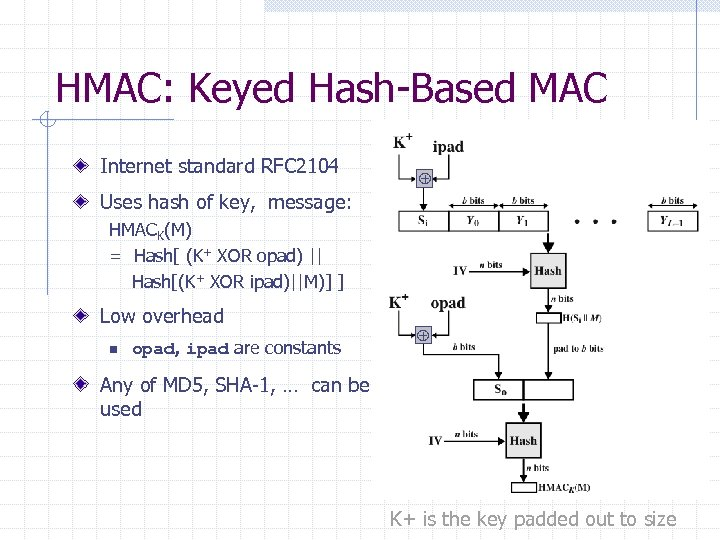HMAC: Keyed Hash-Based MAC Internet standard RFC 2104 Uses hash of key, message: HMACK(M) = Hash[ (K+ XOR opad) || Hash[(K+ XOR ipad)||M)] ] Low overhead n opad, ipad are constants Any of MD 5, SHA-1, … can be used K+ is the key padded out to size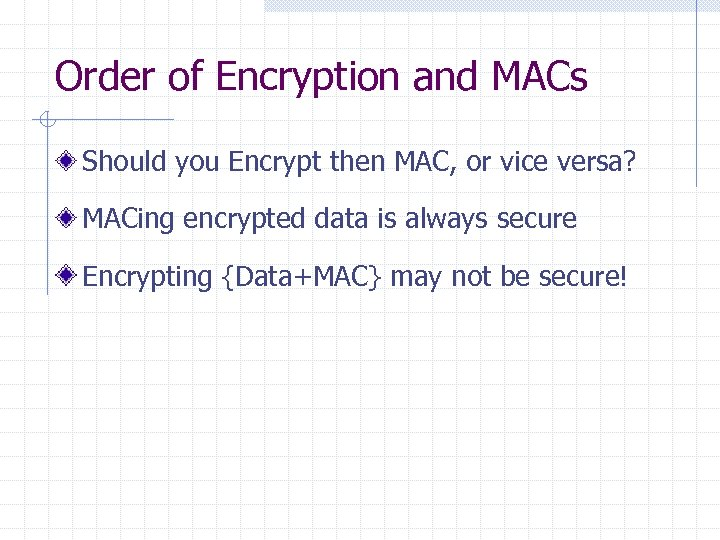Order of Encryption and MACs Should you Encrypt then MAC, or vice versa? MACing encrypted data is always secure Encrypting {Data+MAC} may not be secure!